# Illustrations from the Book

## Chapter 4: Data and their Preparation for CAQDAS Packages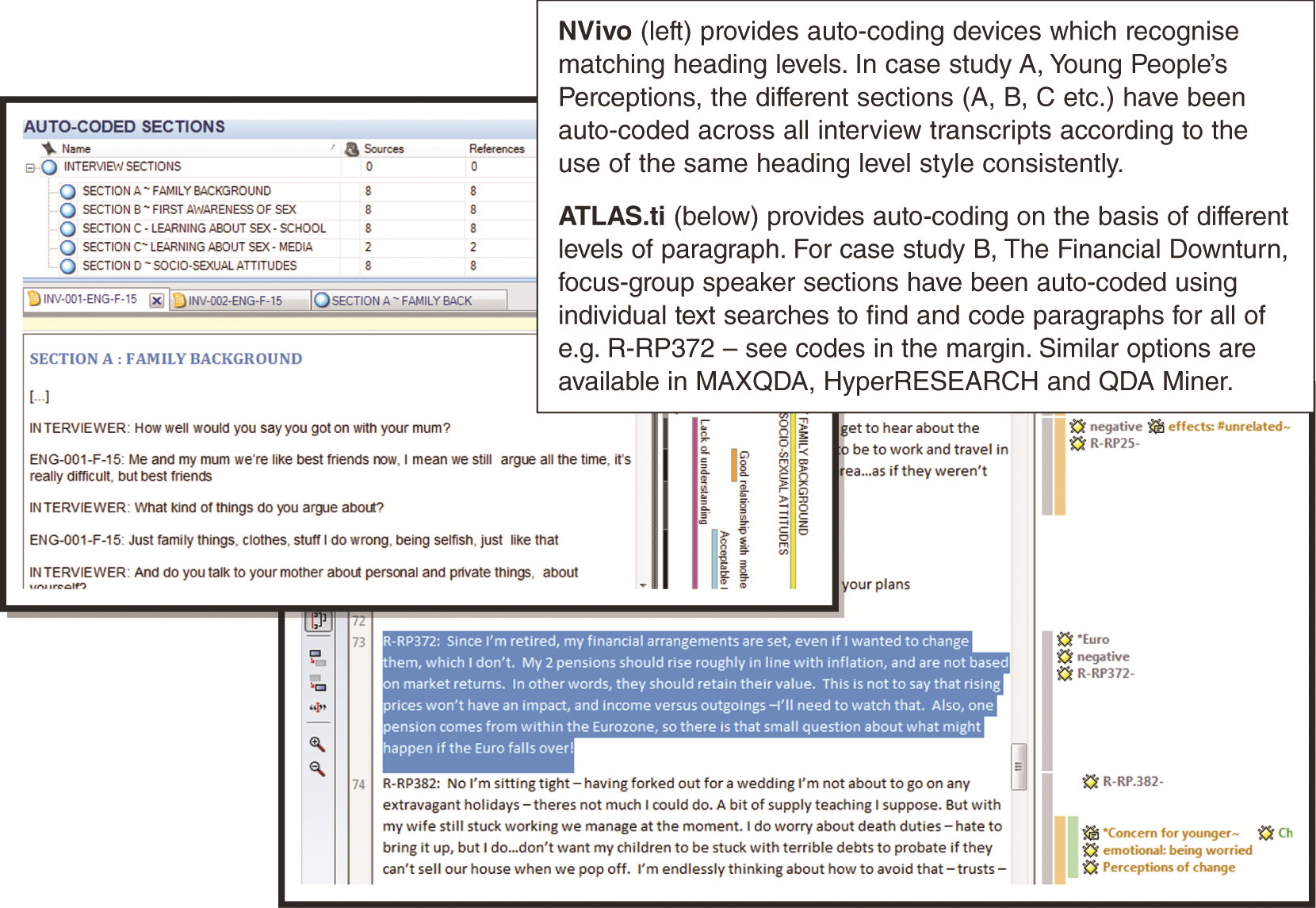Figure 4.1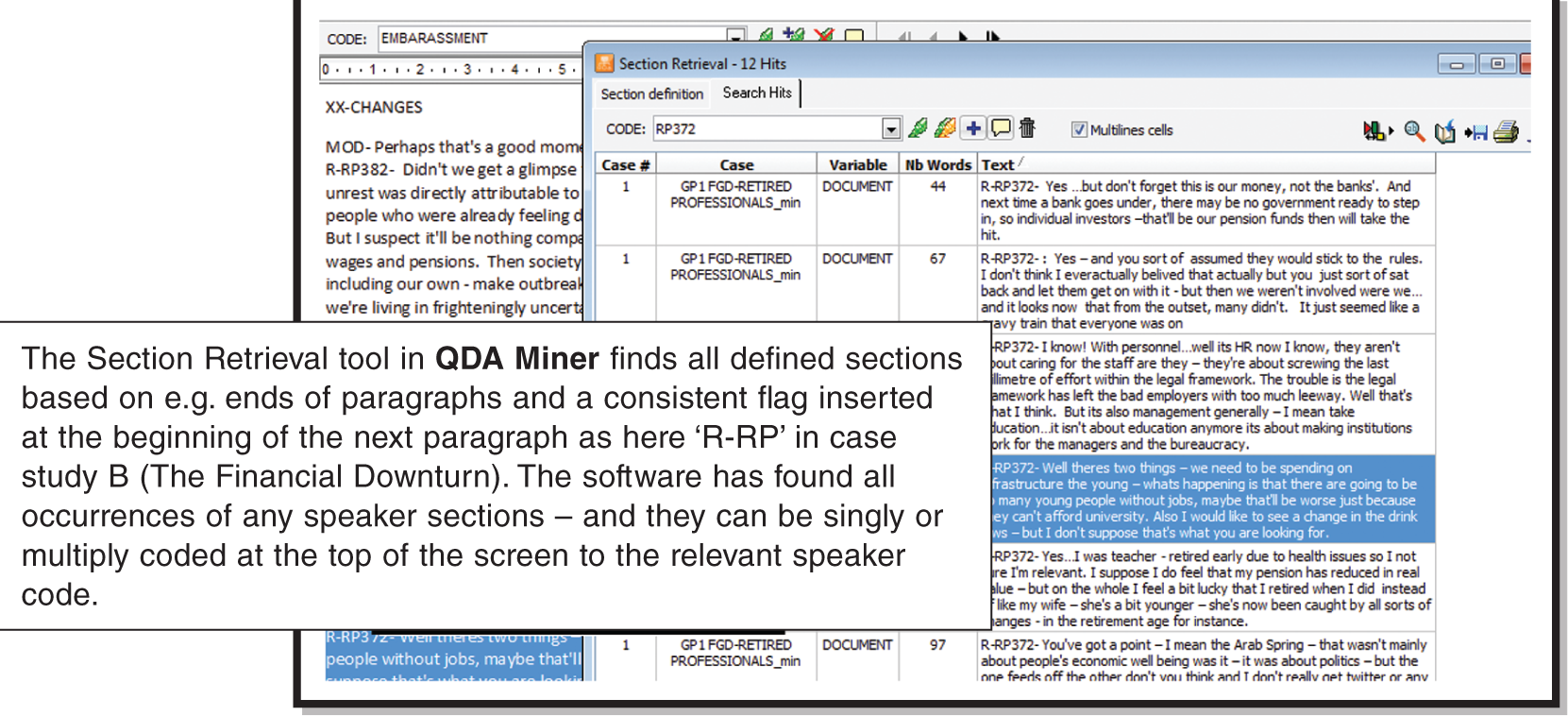Figure 4.2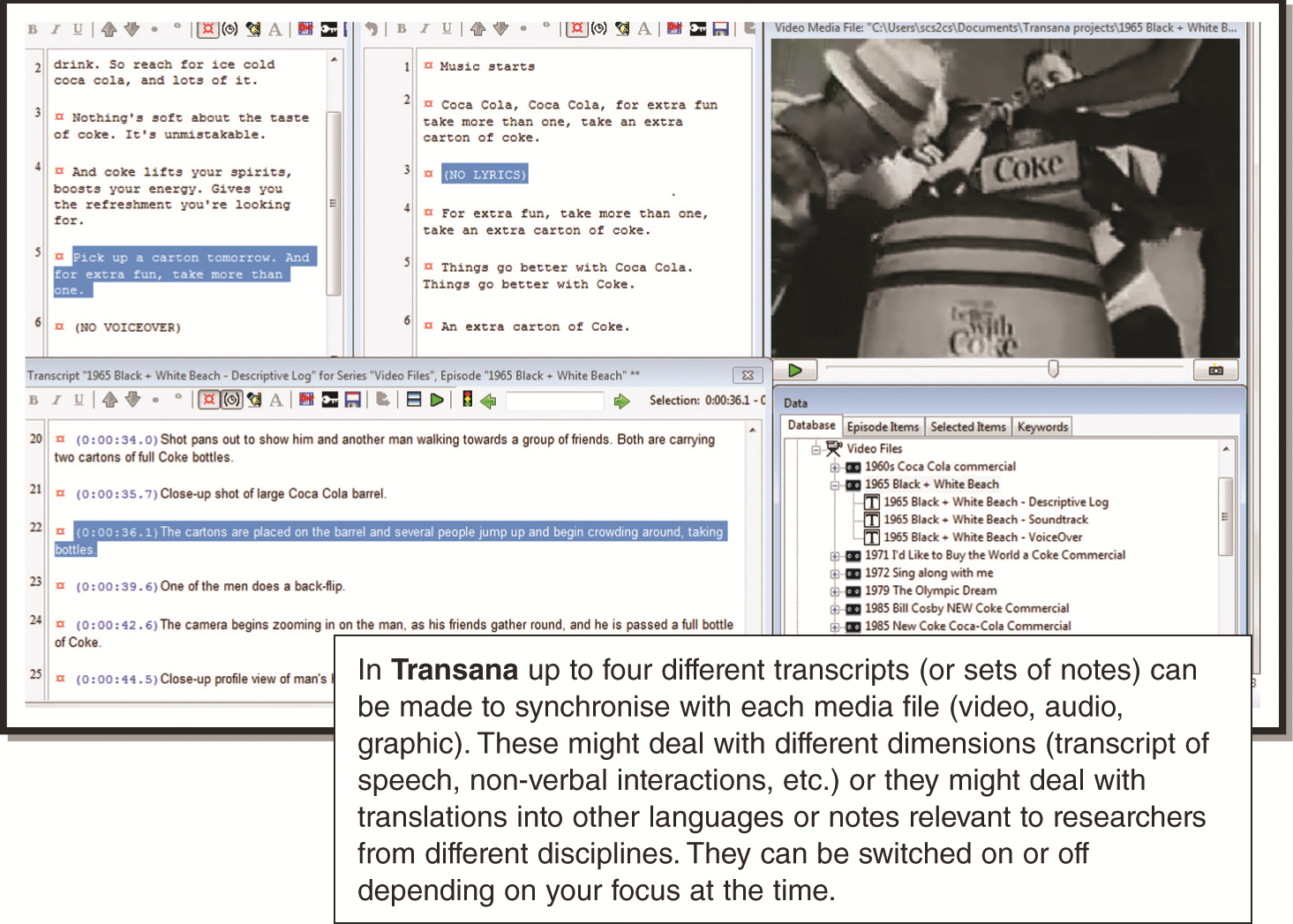Figure 4.3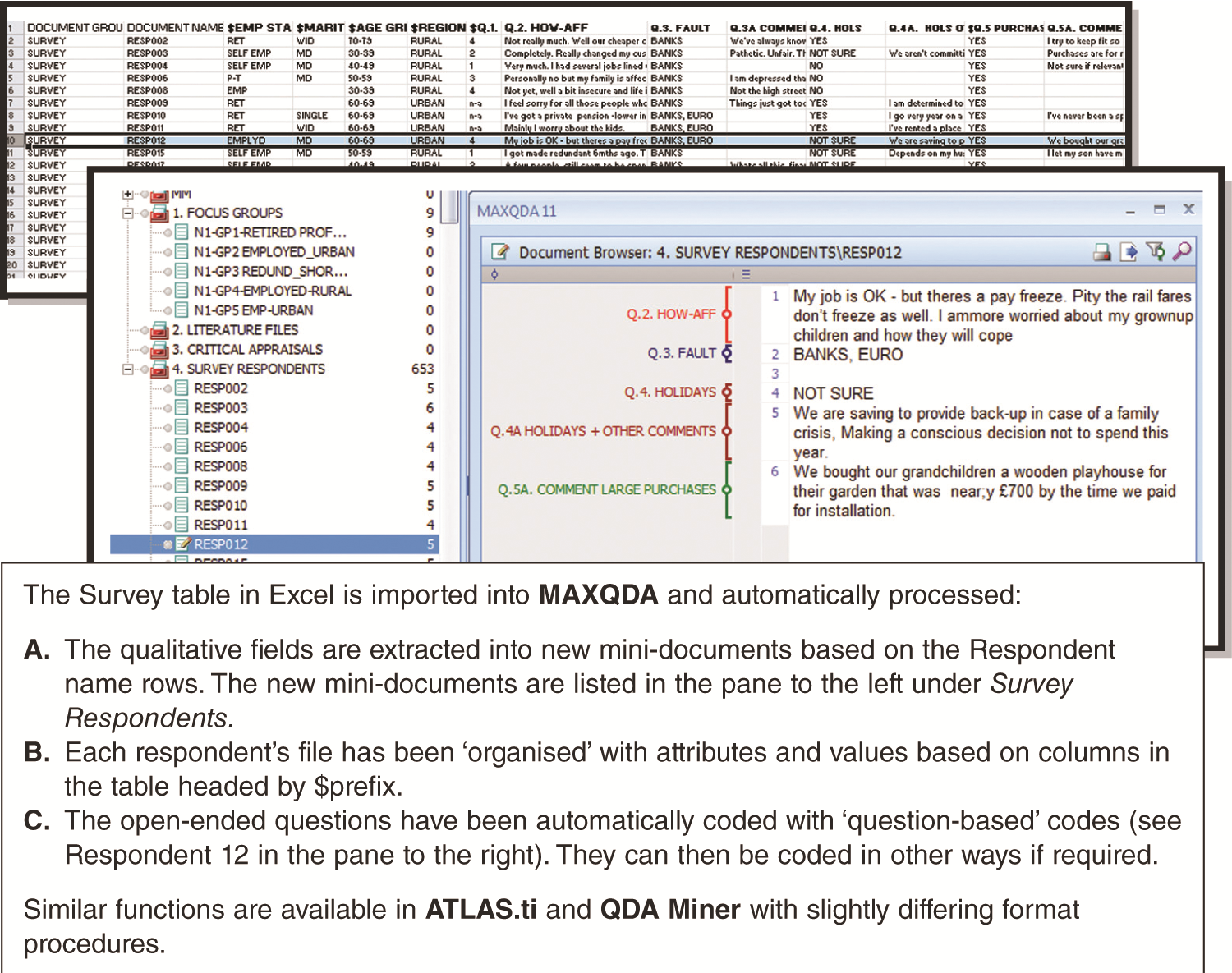Figure 4.4

## Chapter 5: Early Steps in Software: Practical Tasks and FamiliarisationFigure 5.1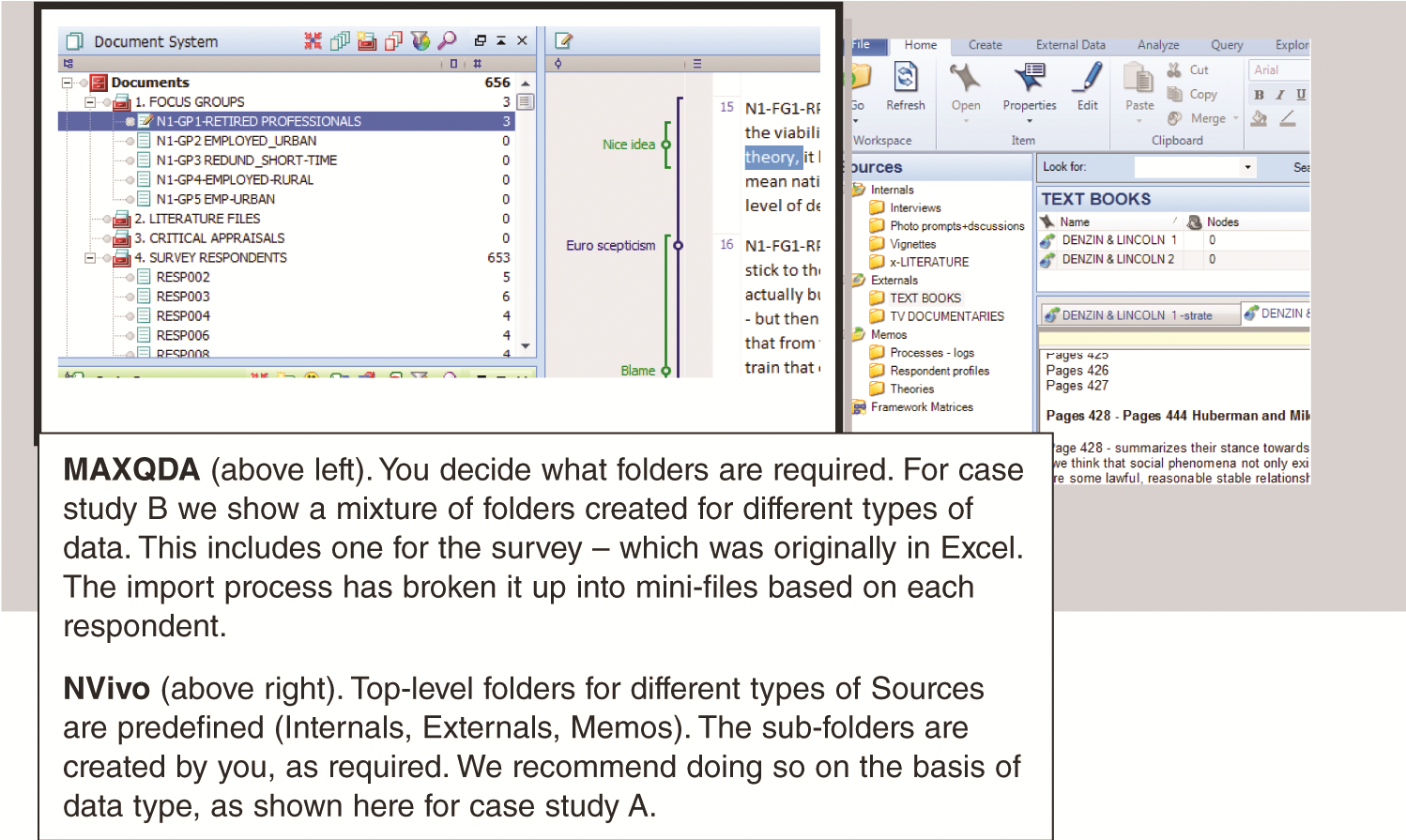Figure 5.2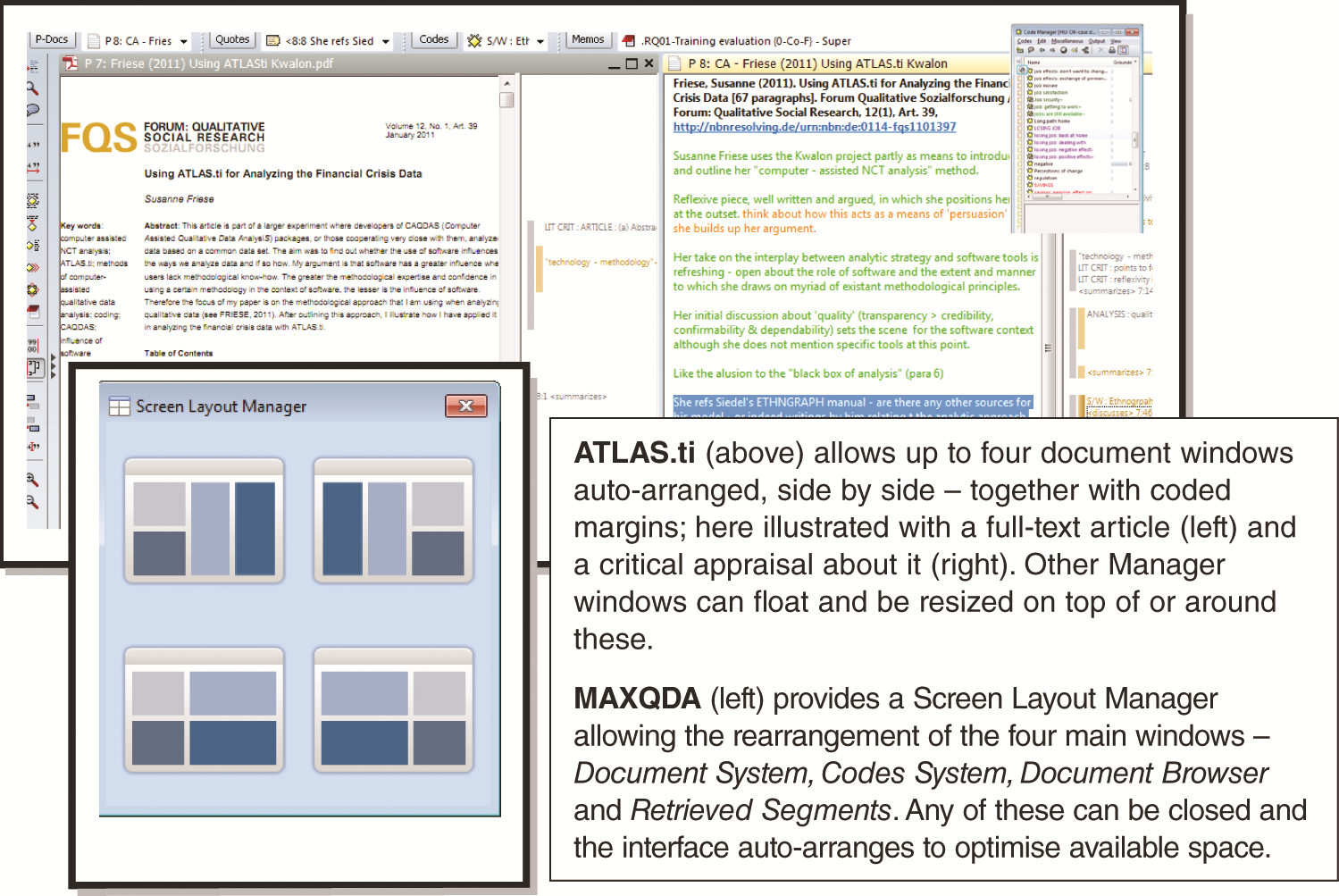Figure 5.3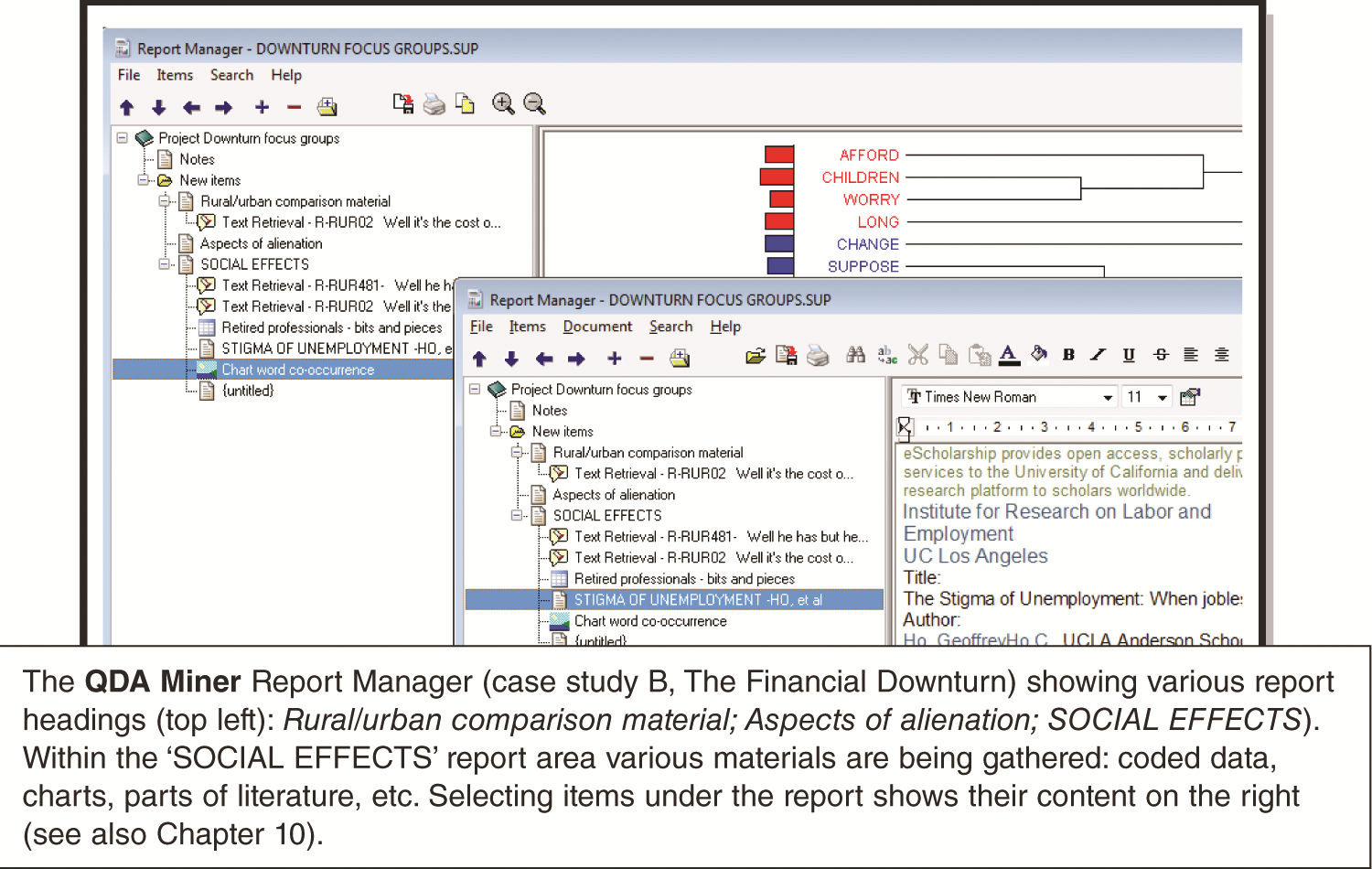Figure 5.4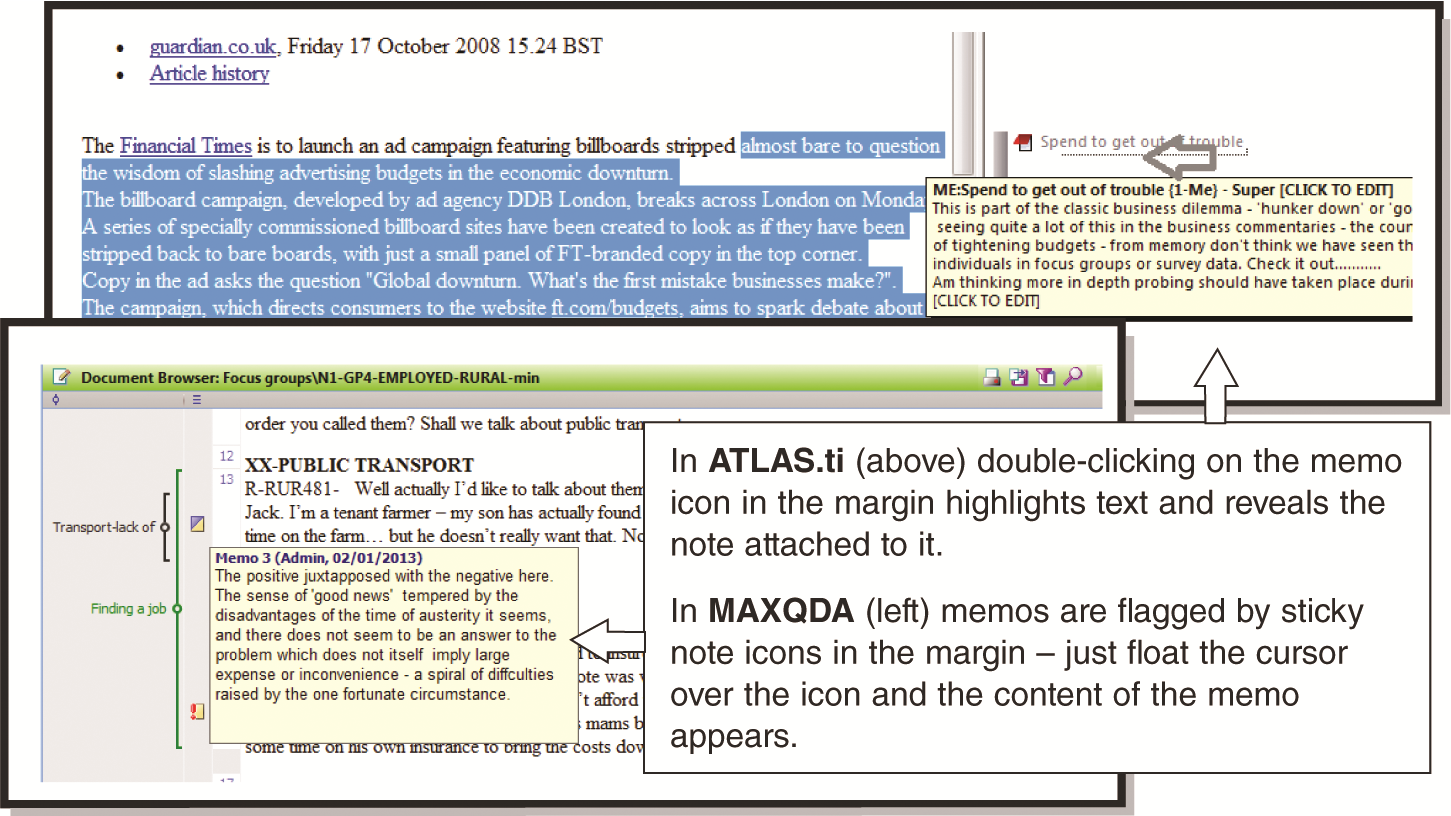Figure 5.5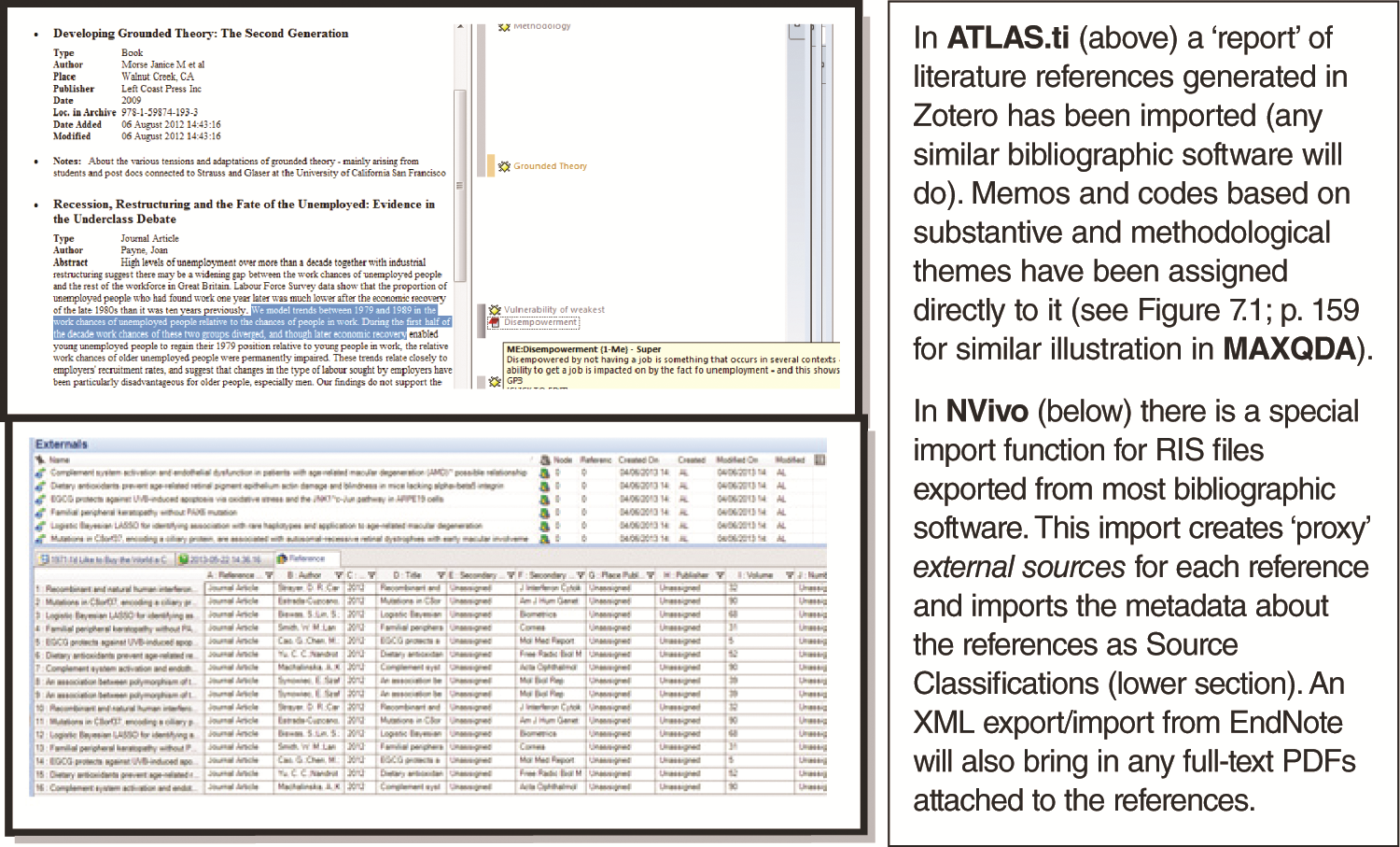Figure 5.6

## Chapter 6: Exploration and Data-level WorkFigure 6.1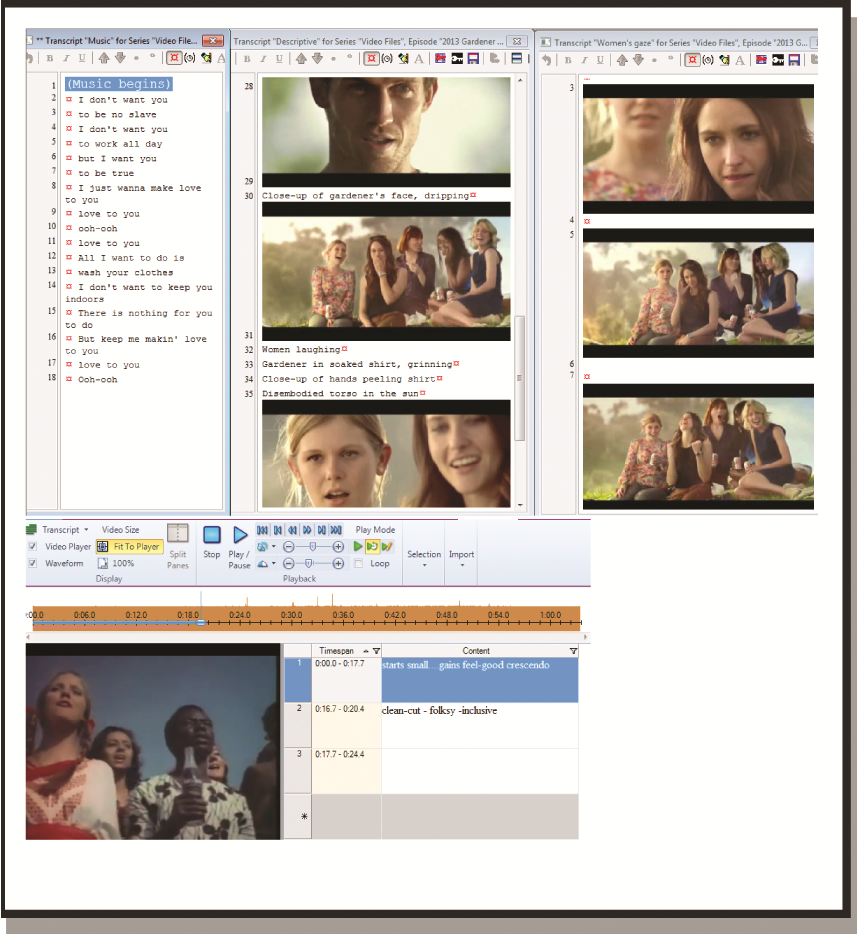Figure 6.2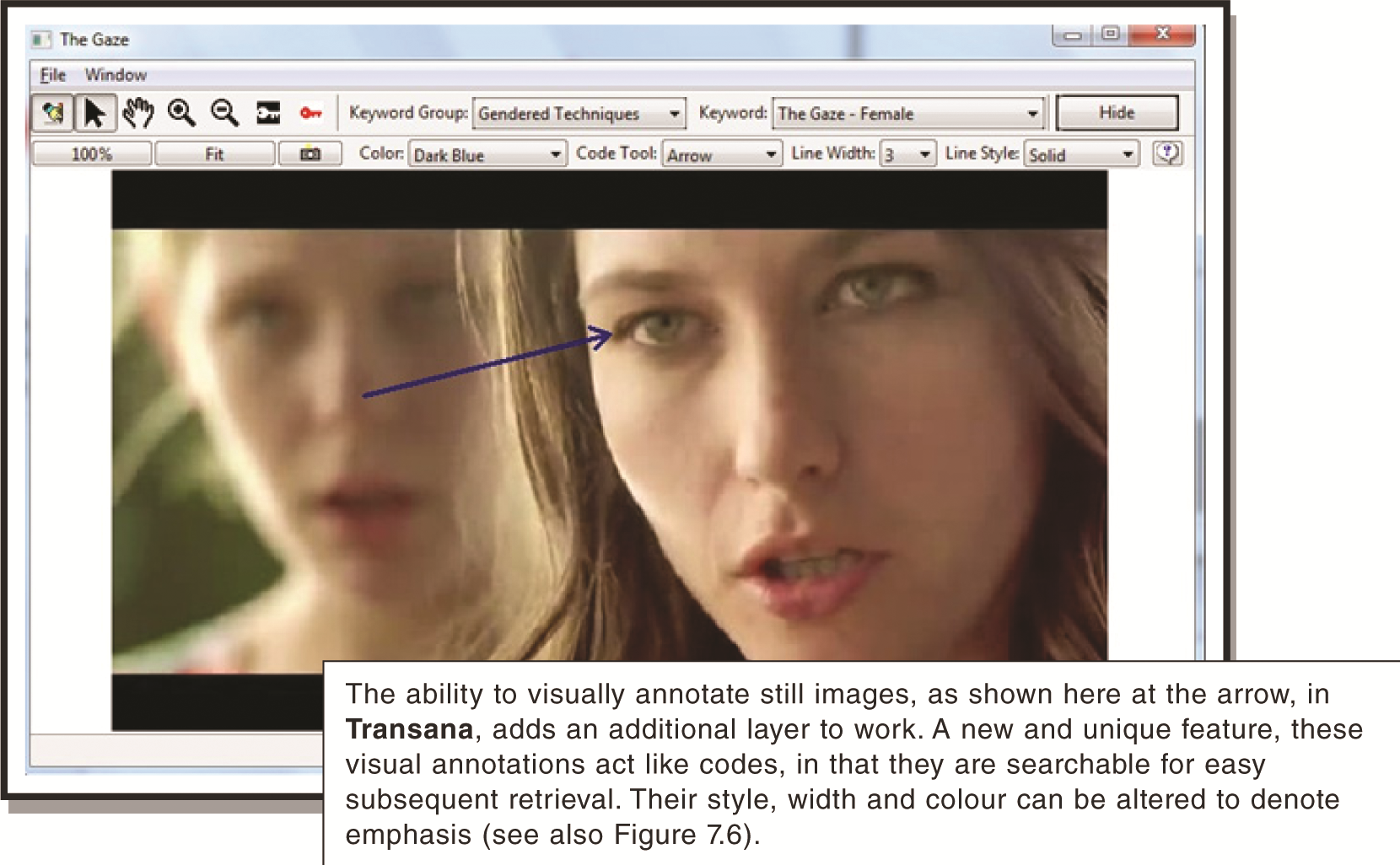Figure 6.3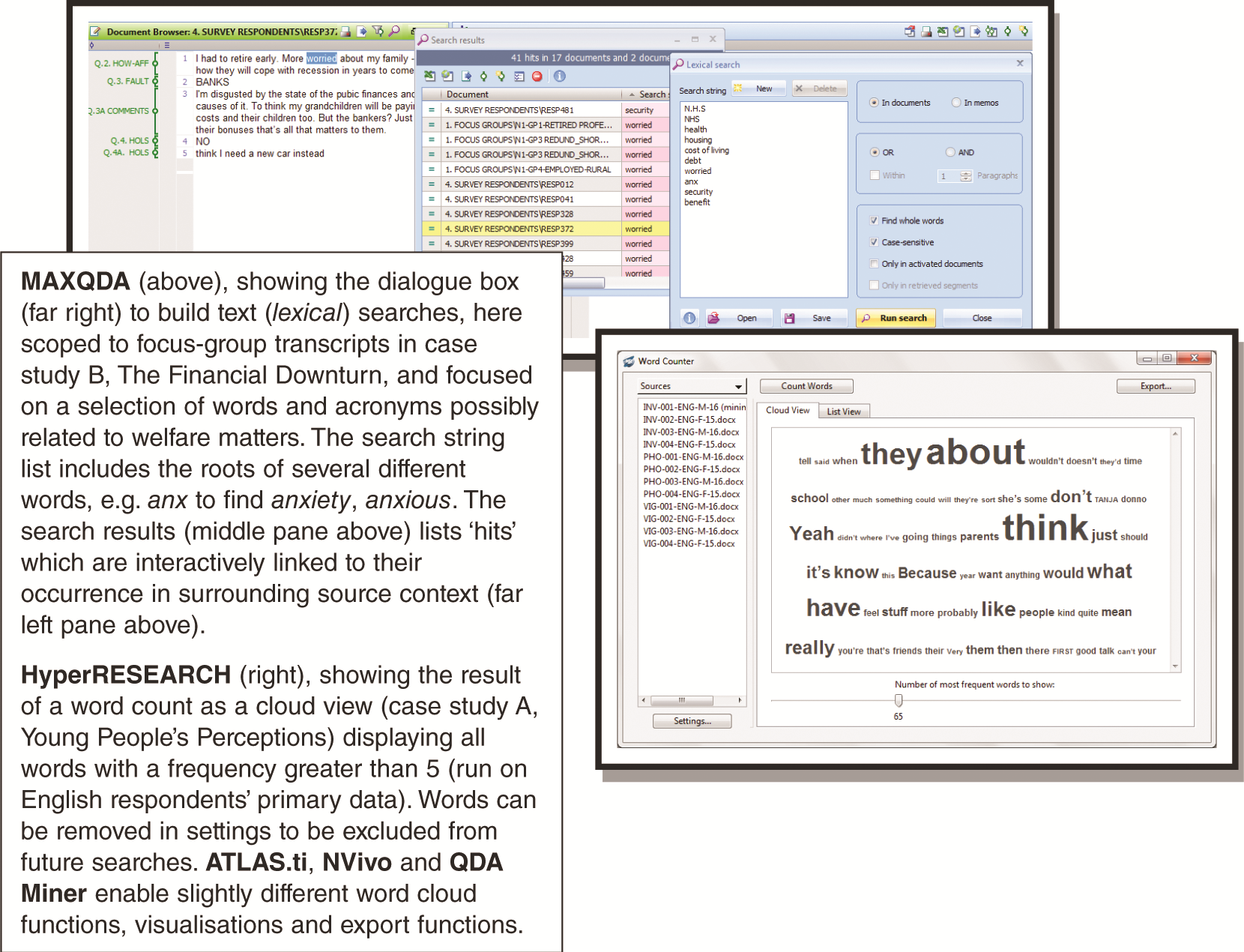Figure 6.4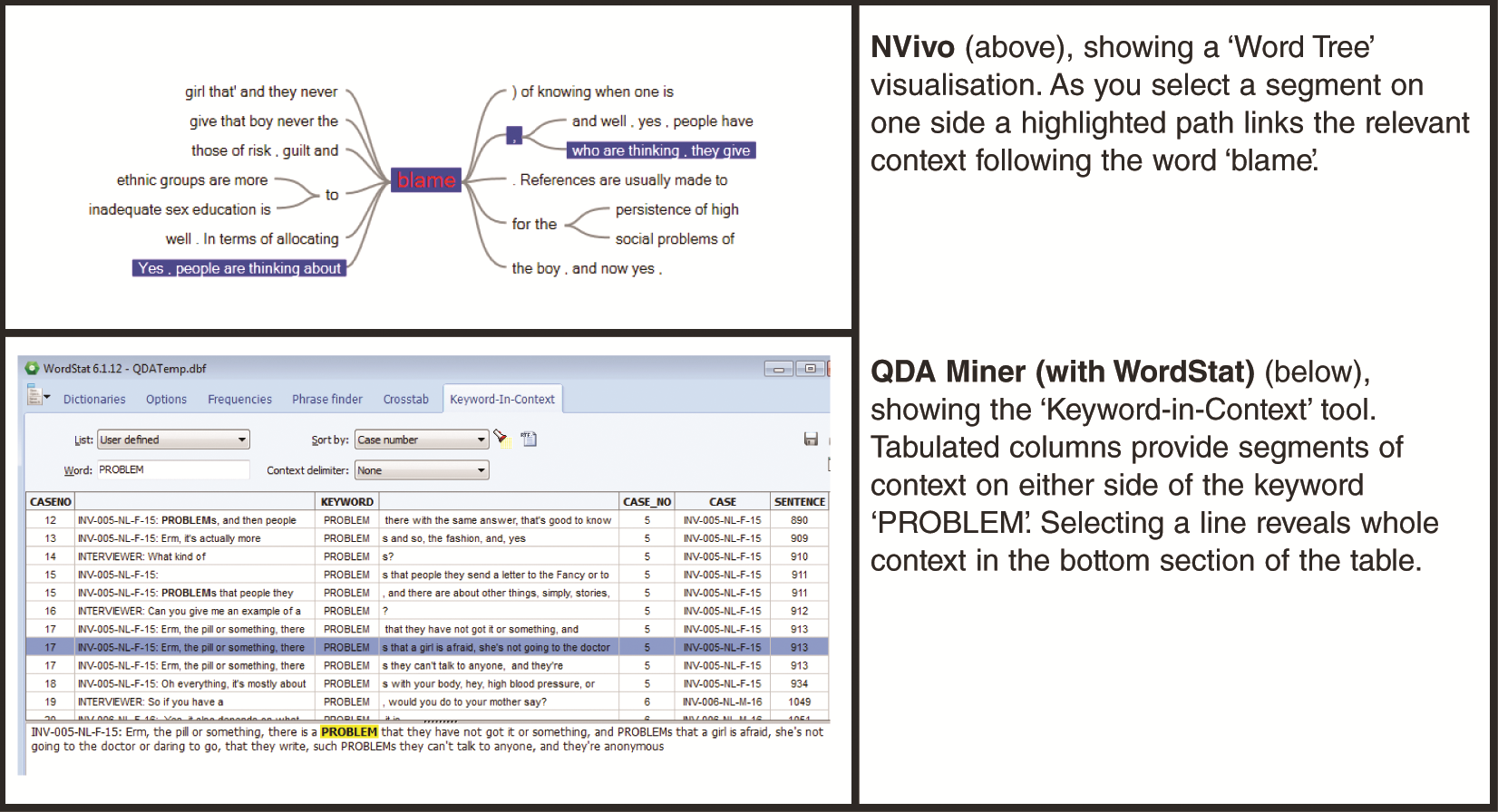Figure 6.5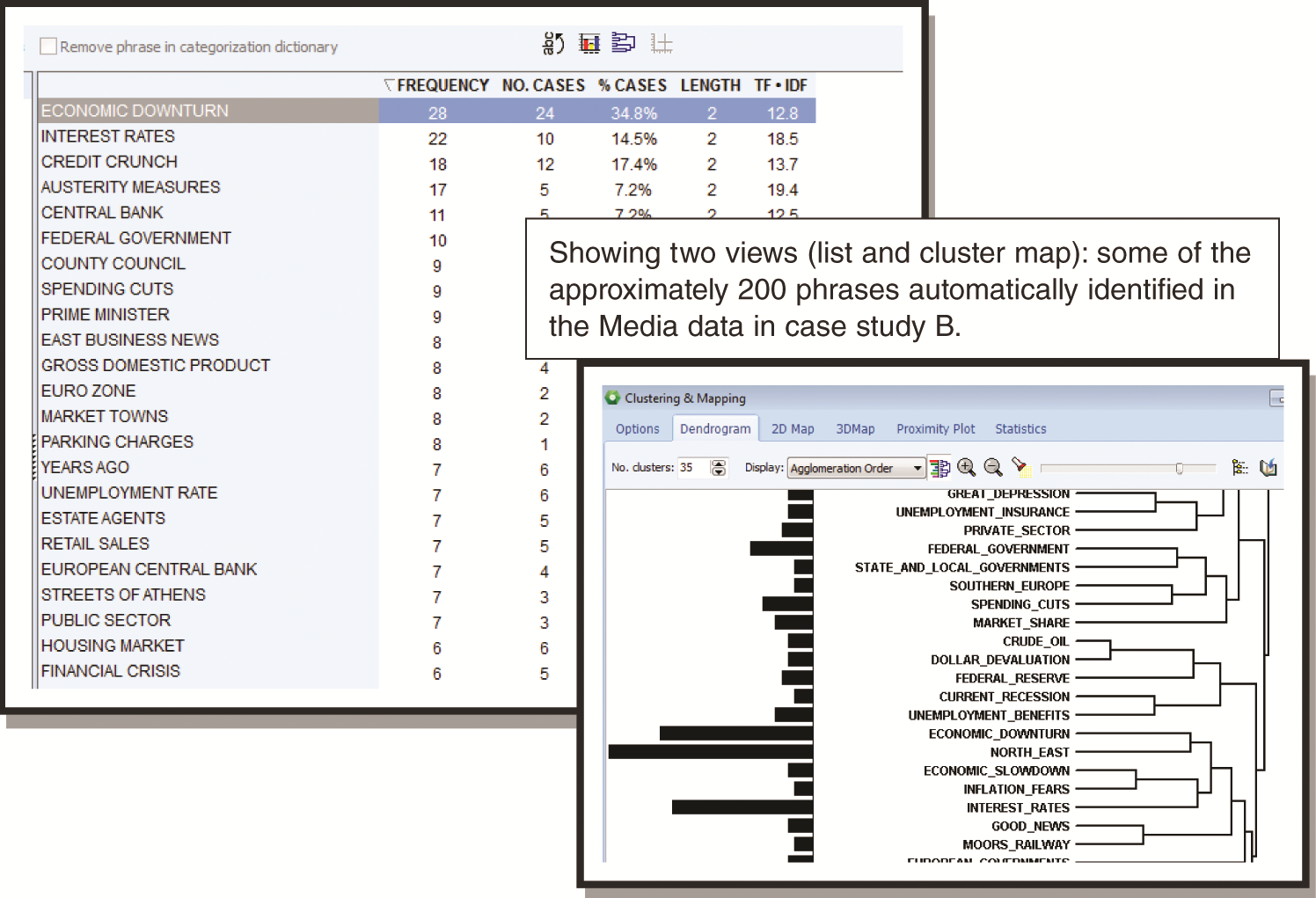Figure 6.6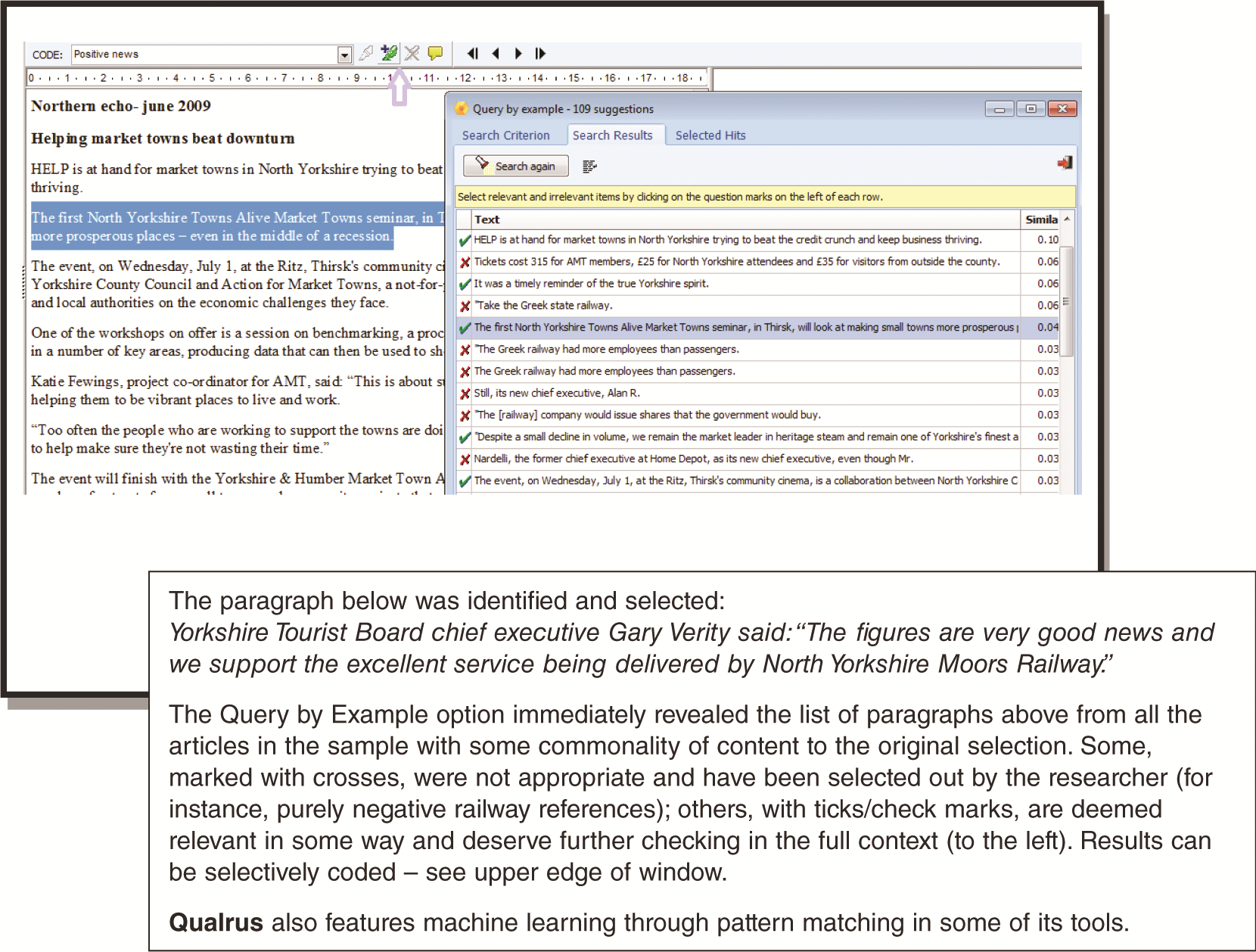Figure 6.7

## Chapter 7: Qualitative Coding in Software: Principles and Processes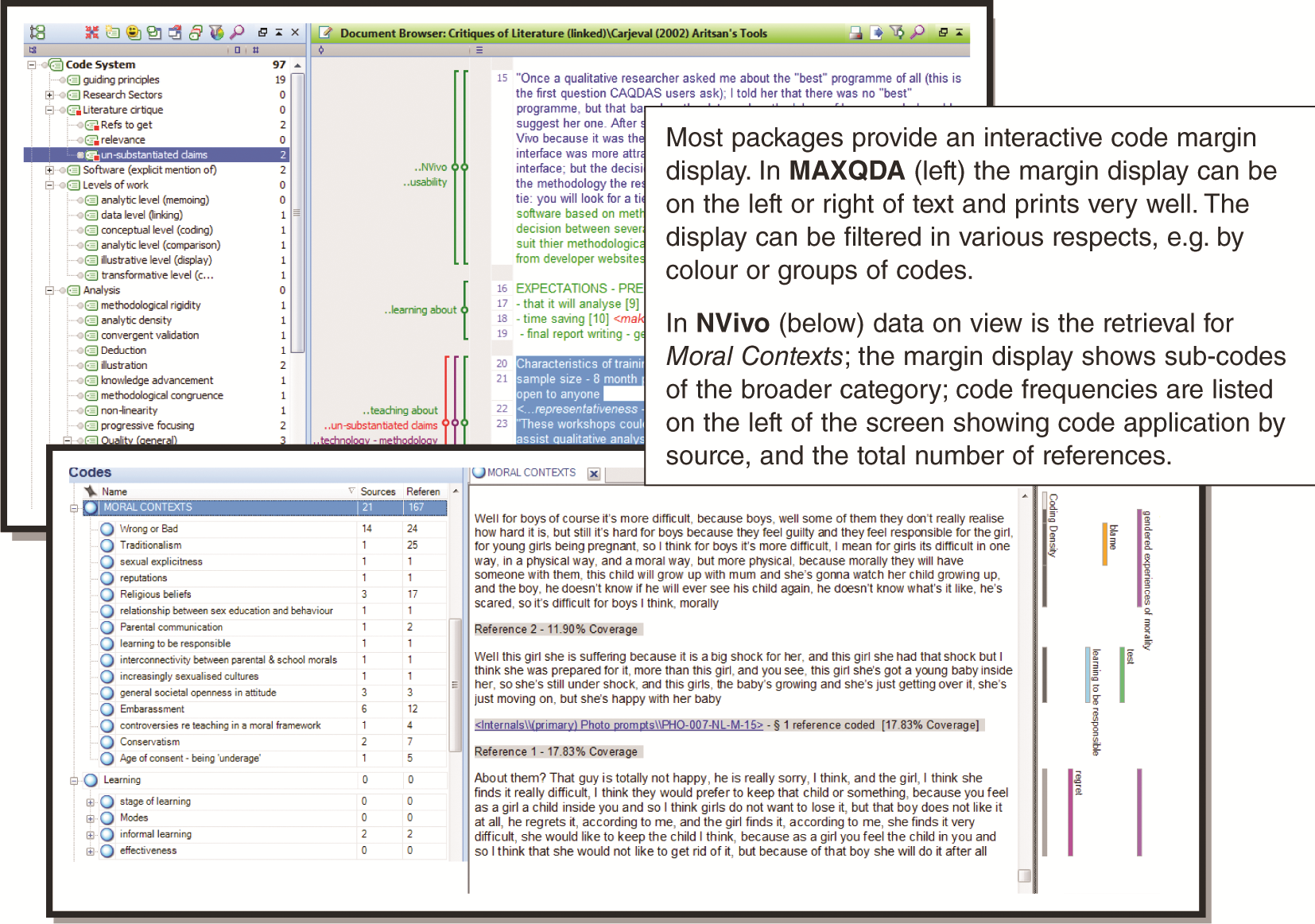Figure 7.1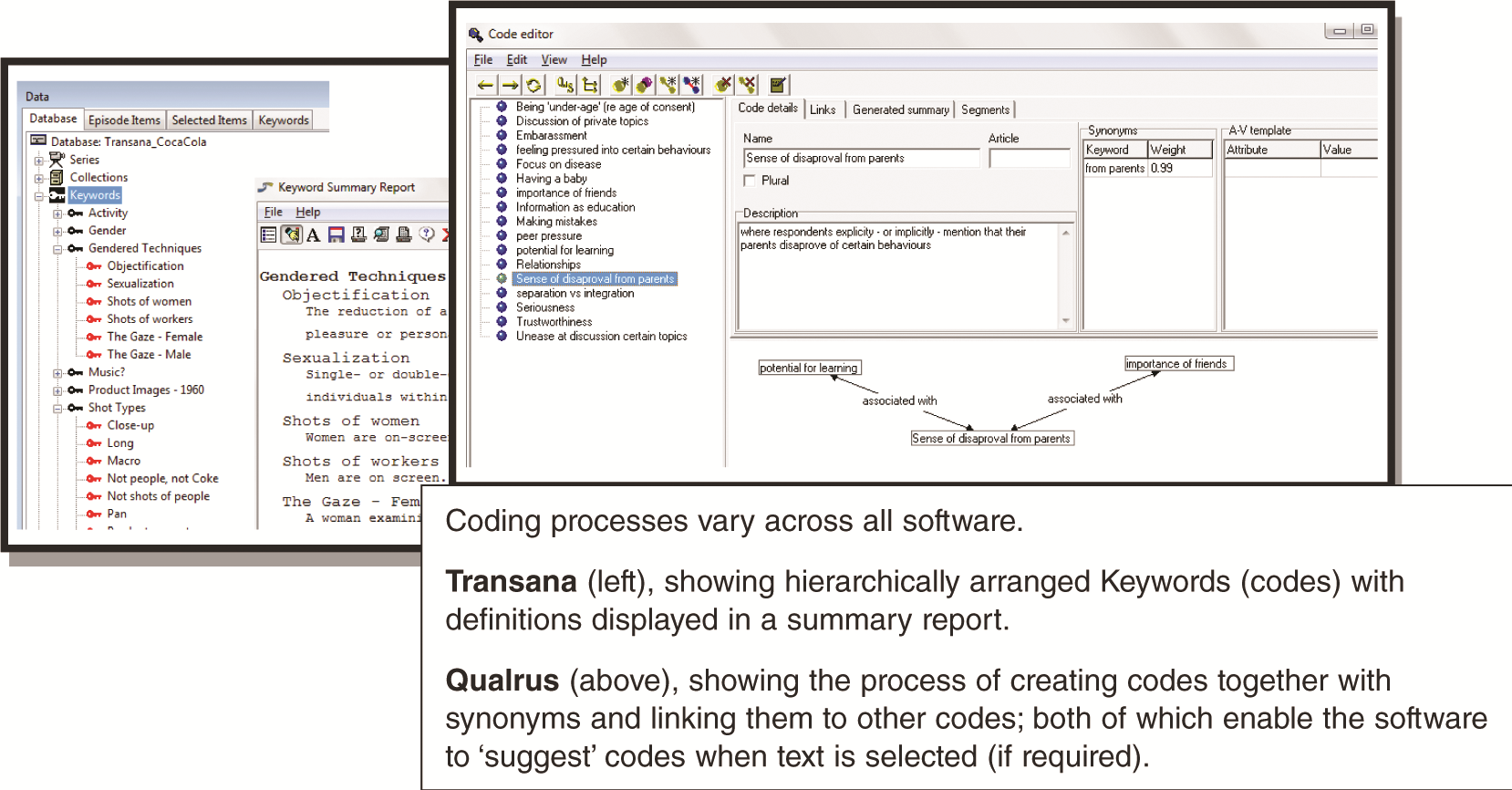Figure 7.2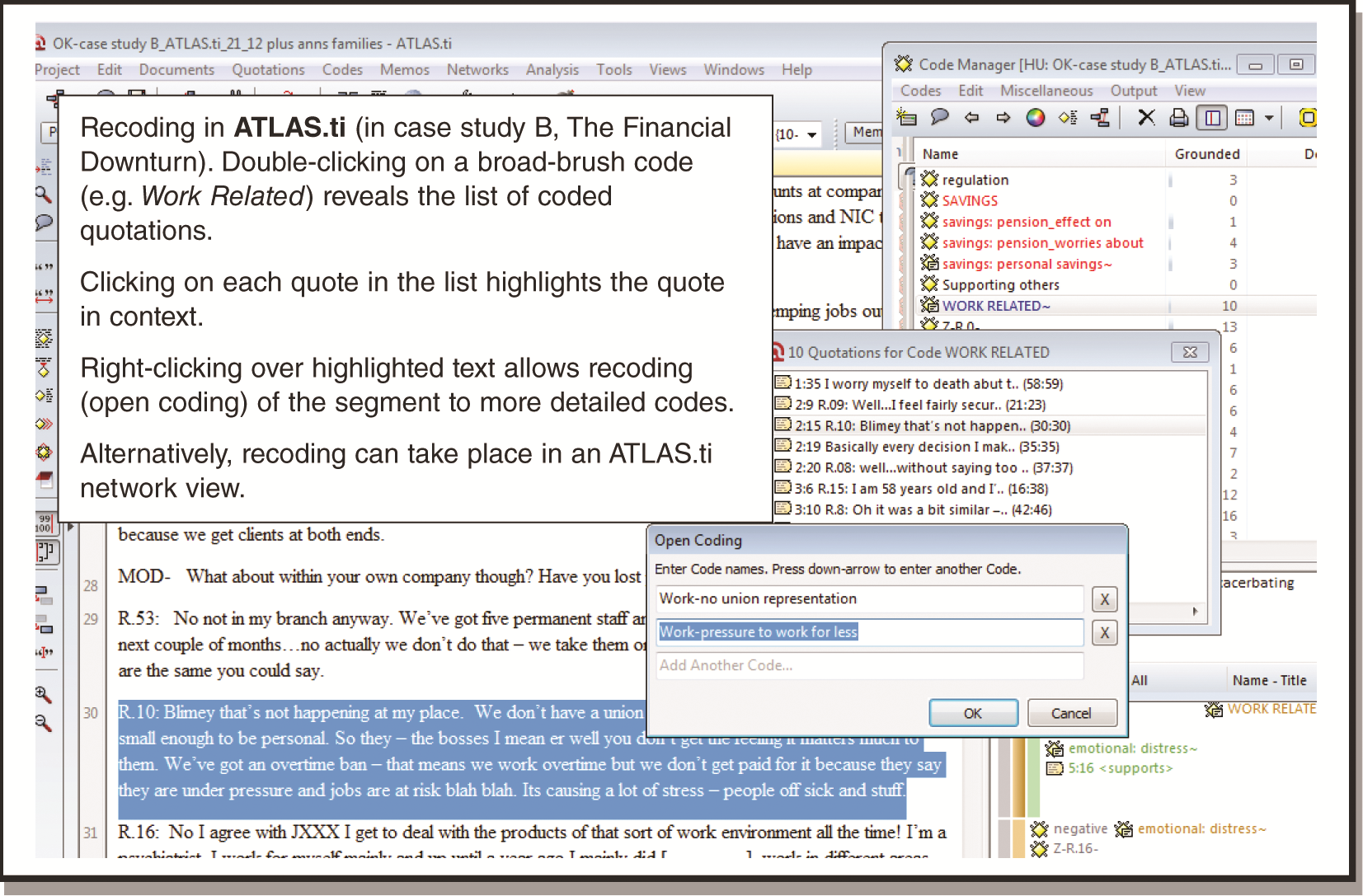Figure 7.3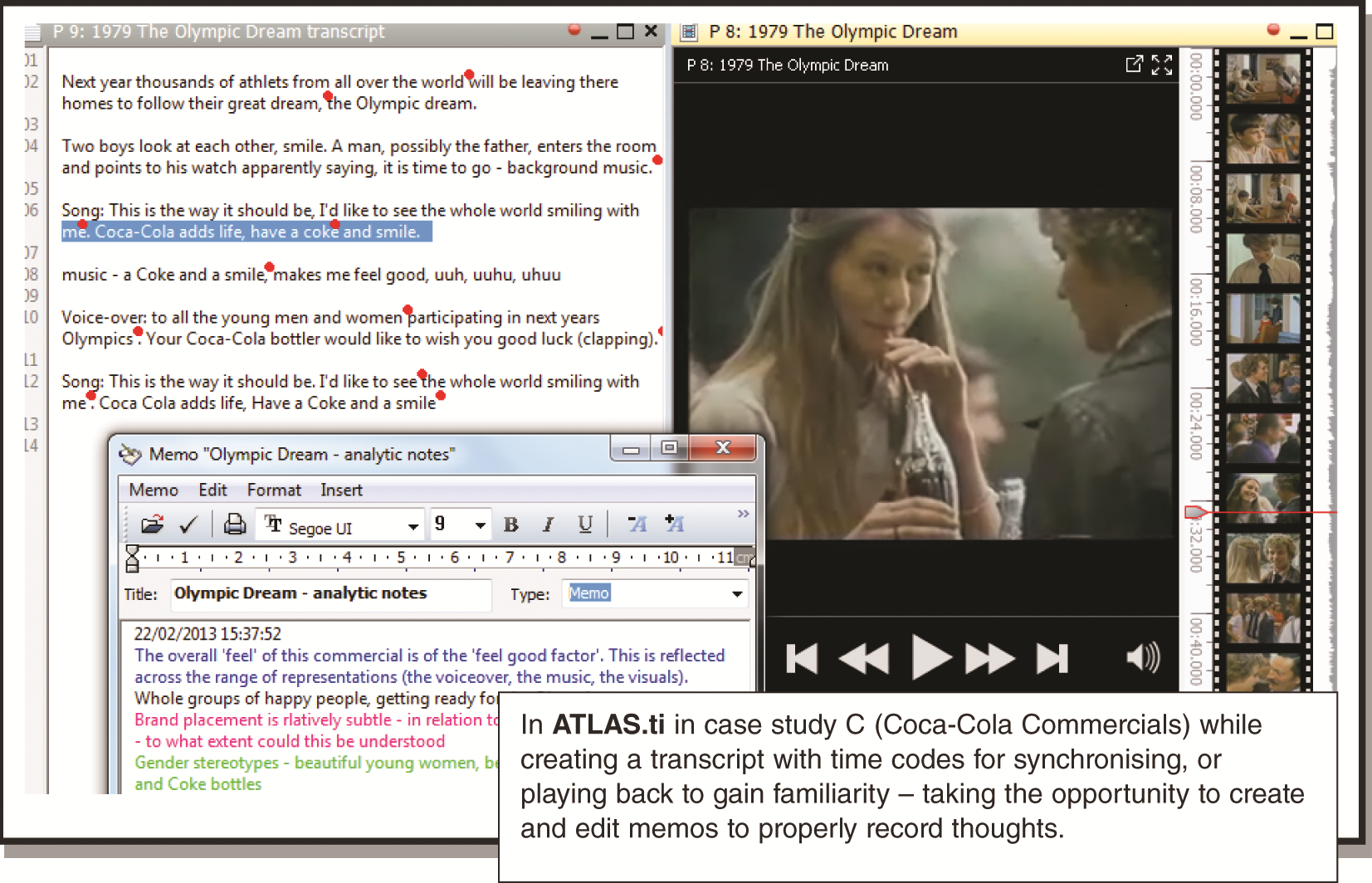Figure 7.4Figure 7.5Figure 7.6

## Chapter 8: Basic Retrieval of Coded Data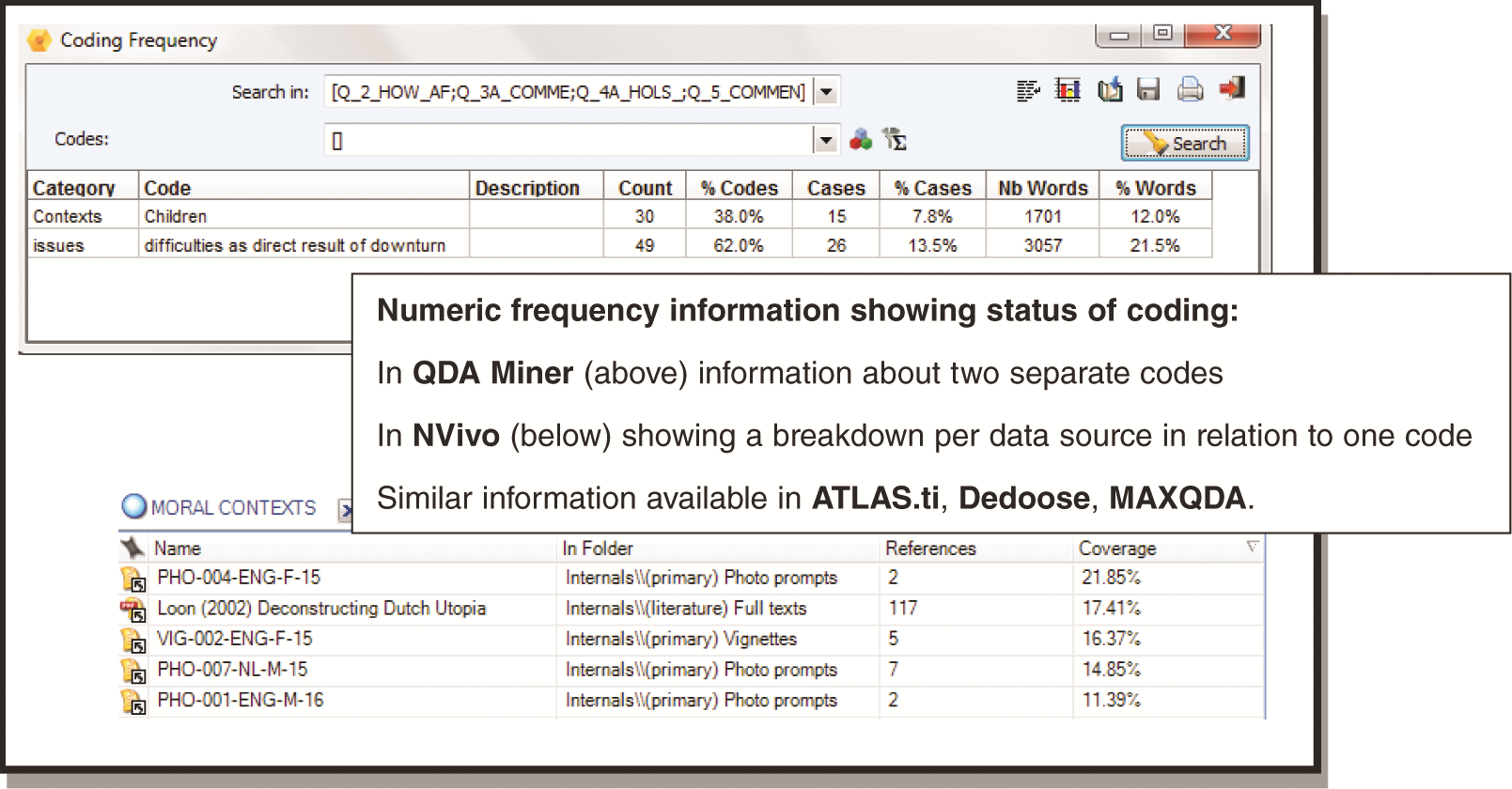Figure 8.1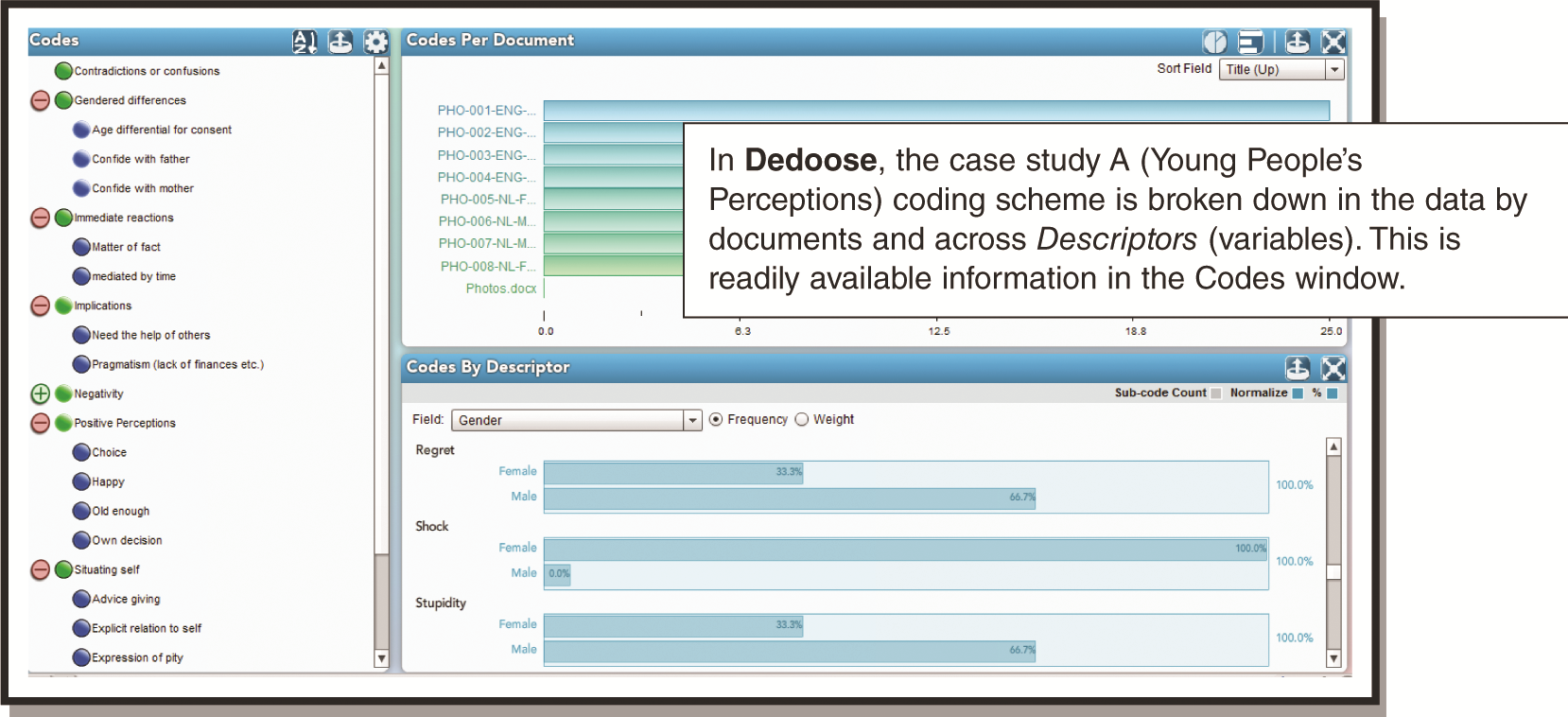Figure 8.2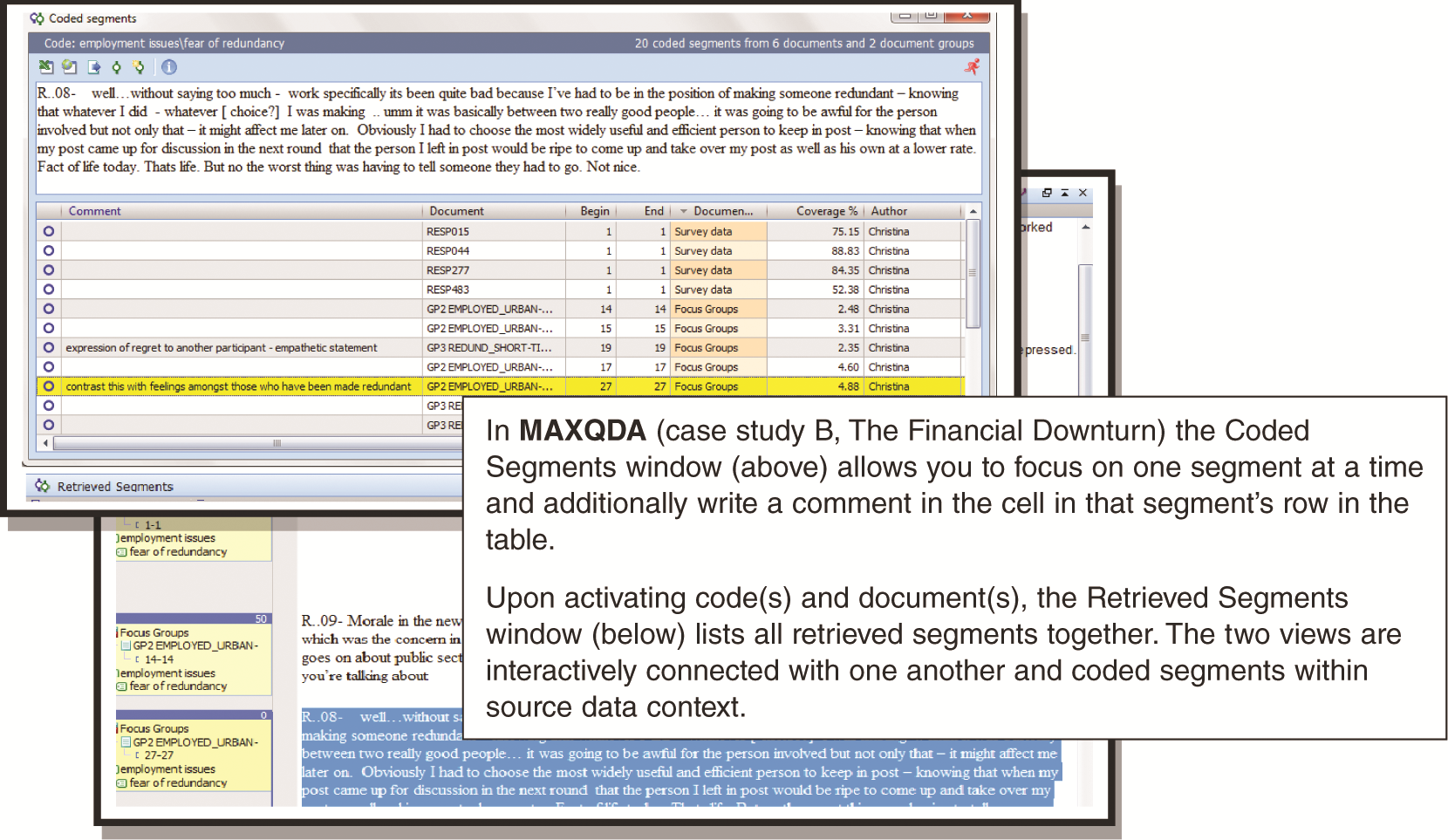Figure 8.3Figure 8.4Figure 8.5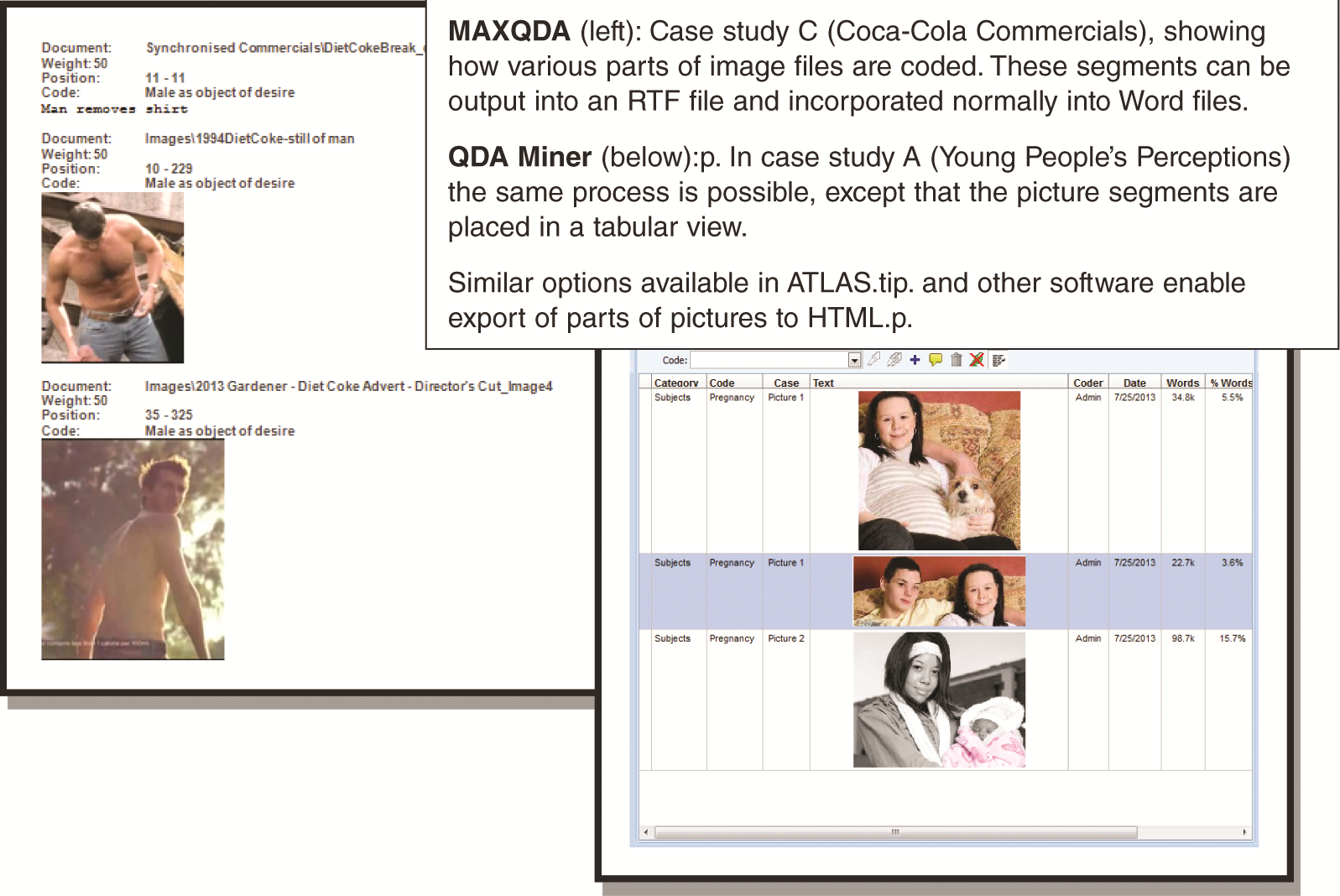Figure 8.6

## Chapter 9: Working with Coding Schemes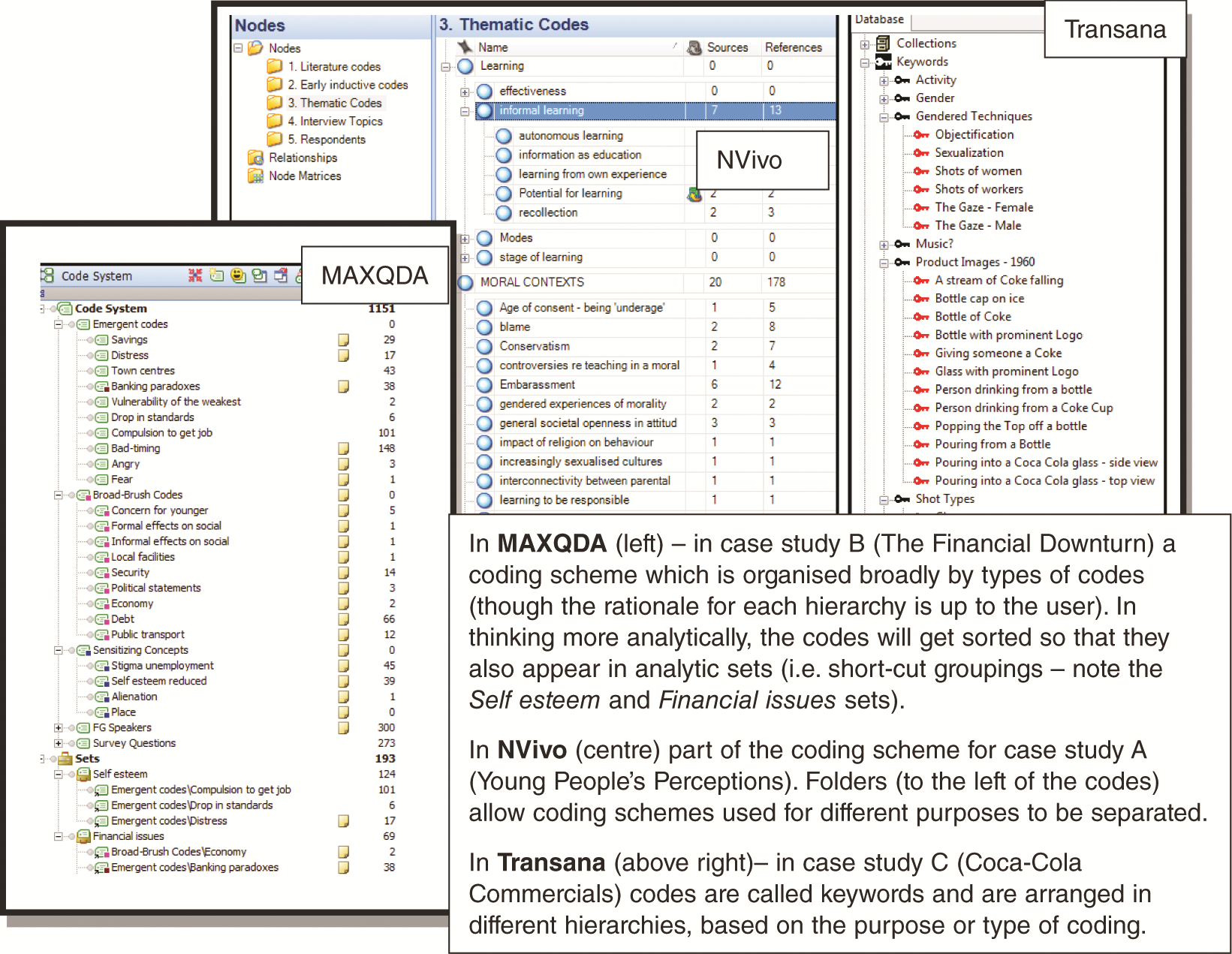Figure 9.1Figure 9.2Figure 9.3Figure 9.4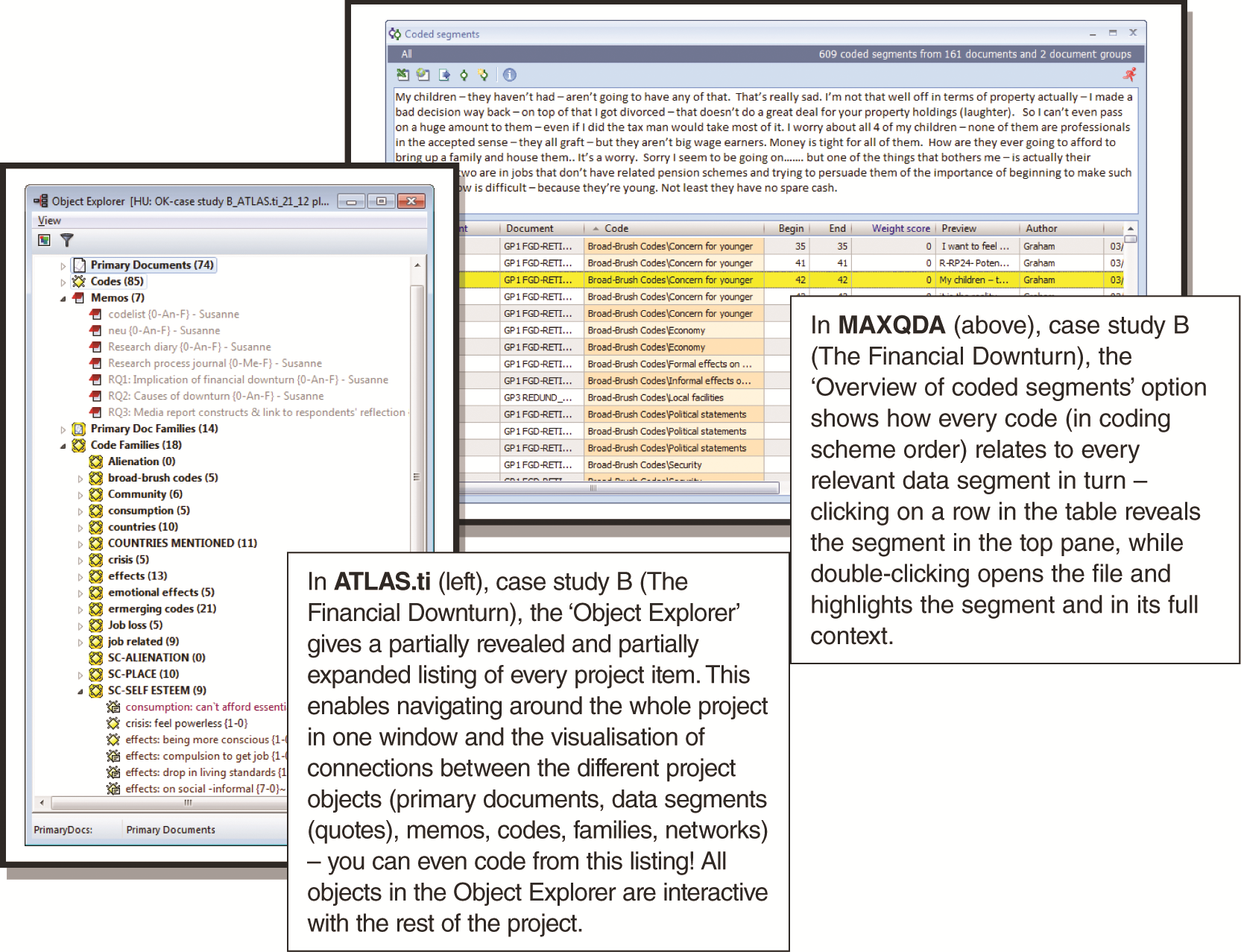Figure 9.5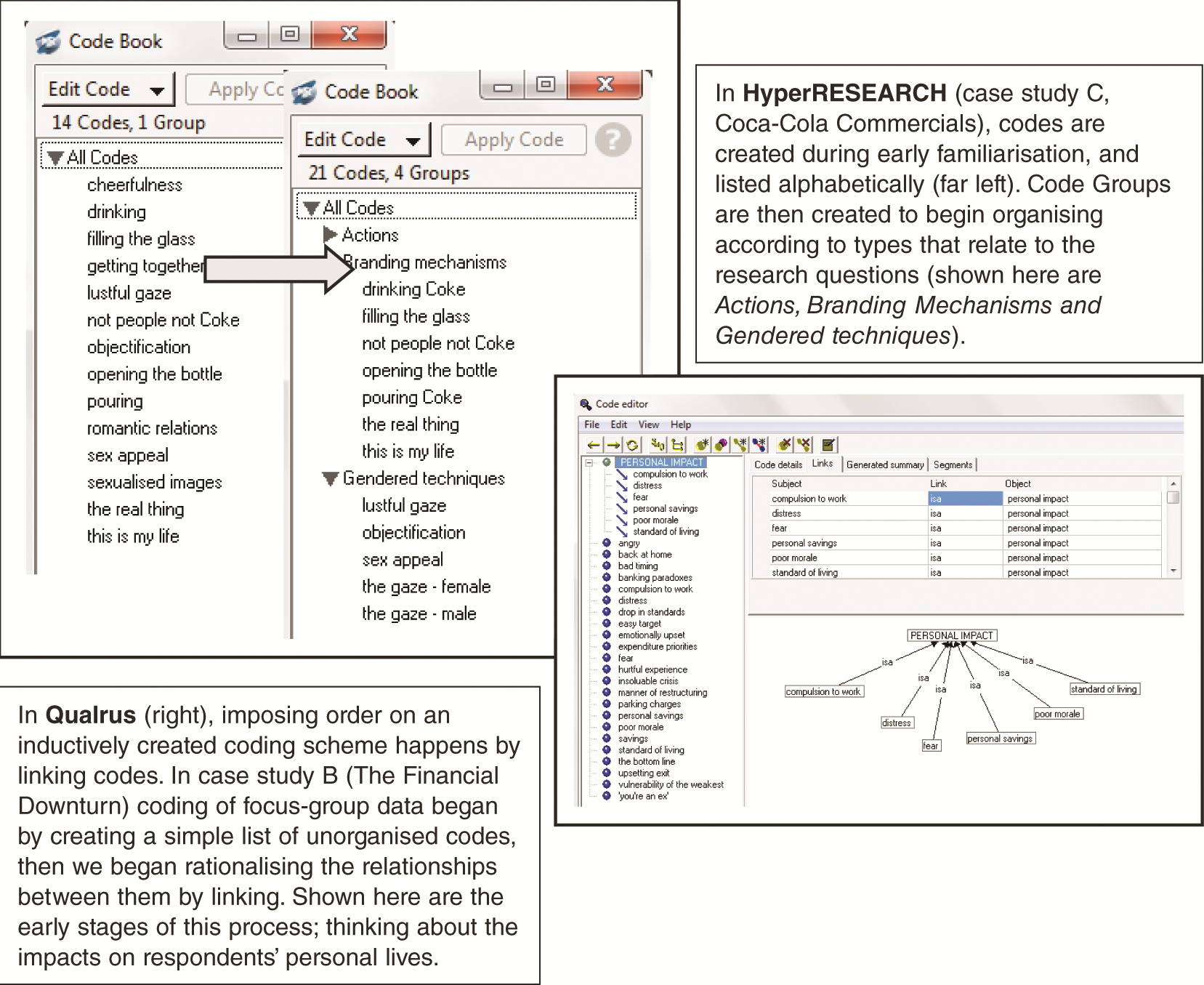Figure 9.6

## Chapter 10: Managing Processes and Interpretations by Writing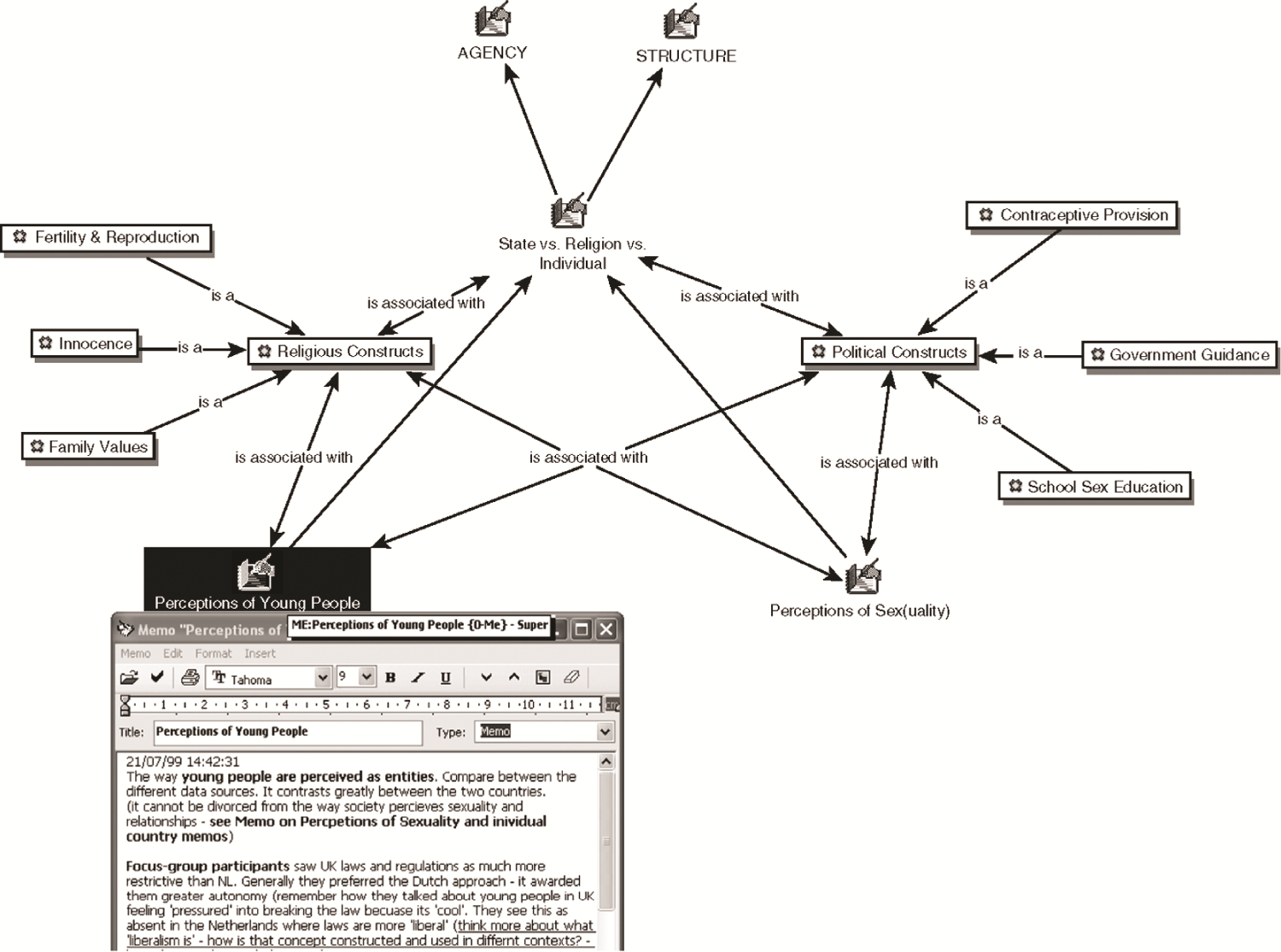Figure 10.1Figure 10.2Figure 10.3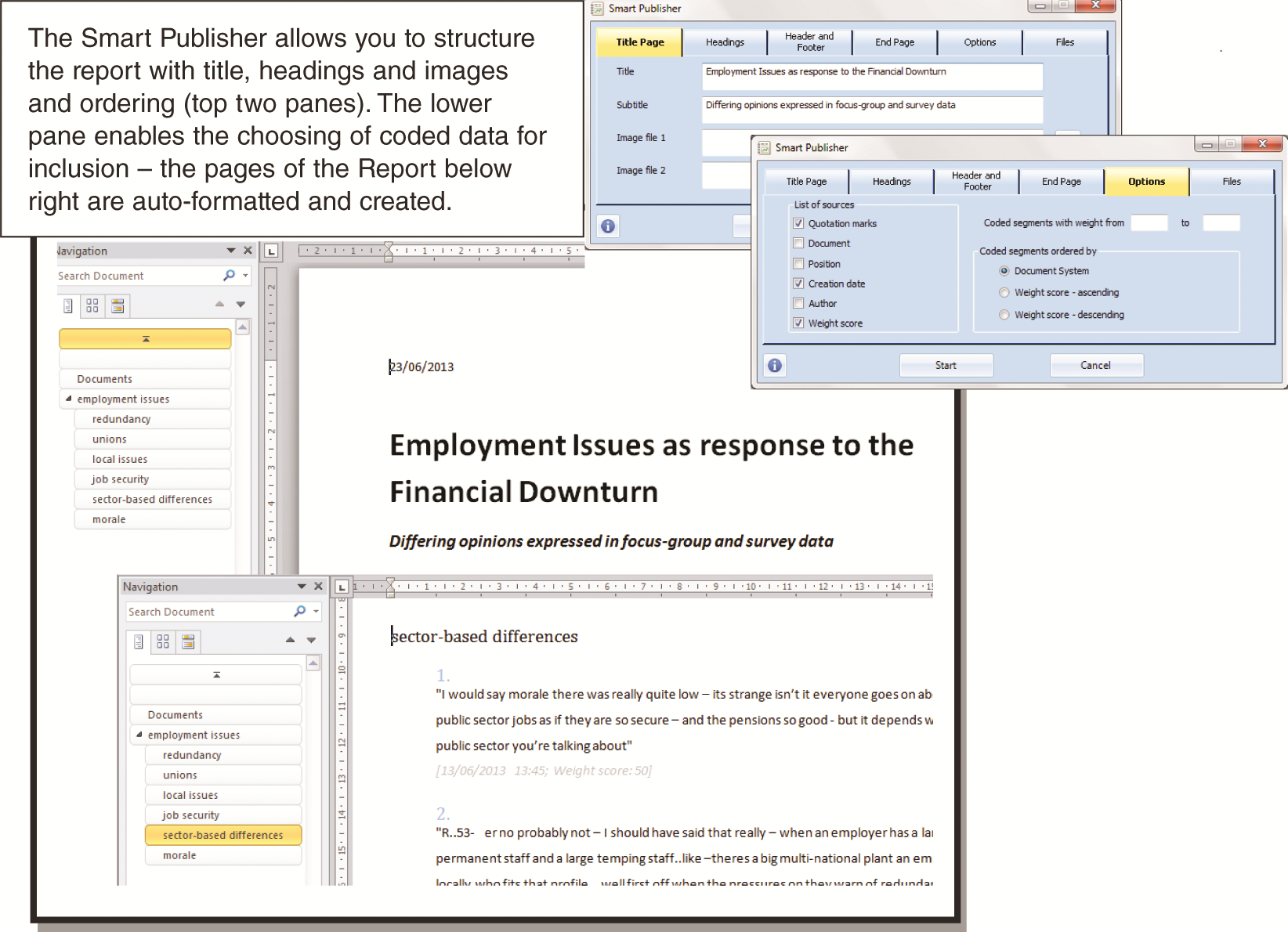Figure 10.4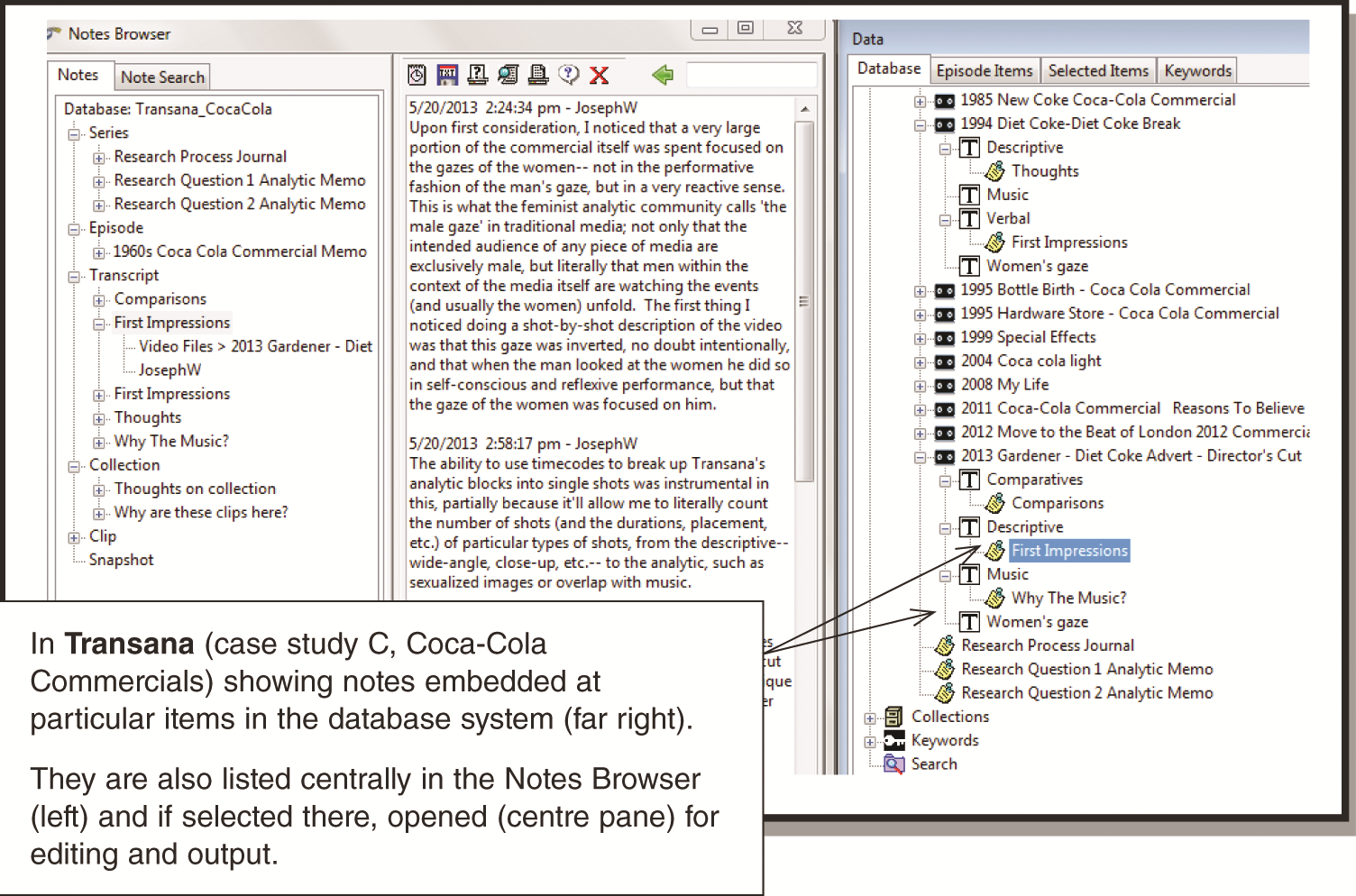Figure 10.5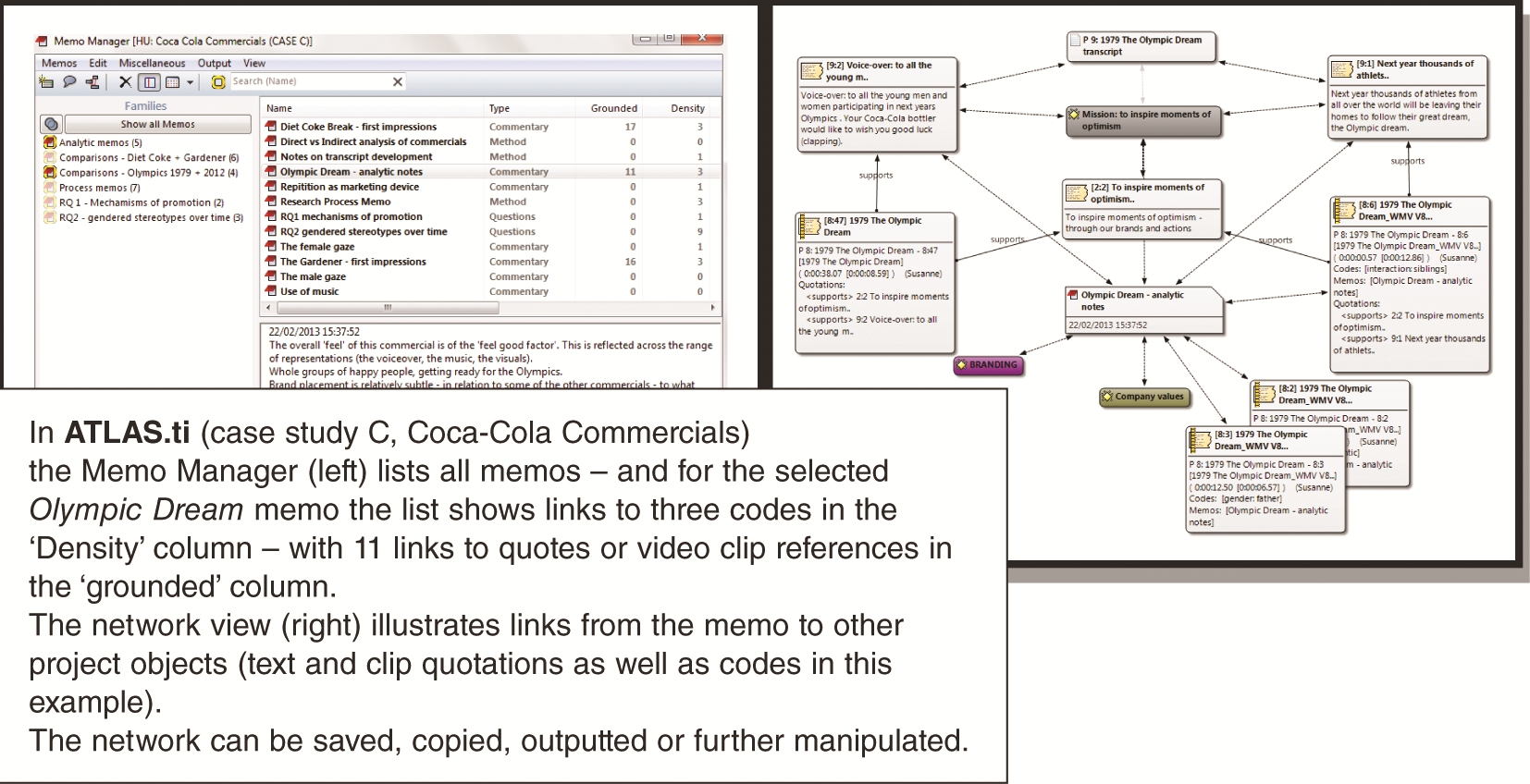Figure 10.6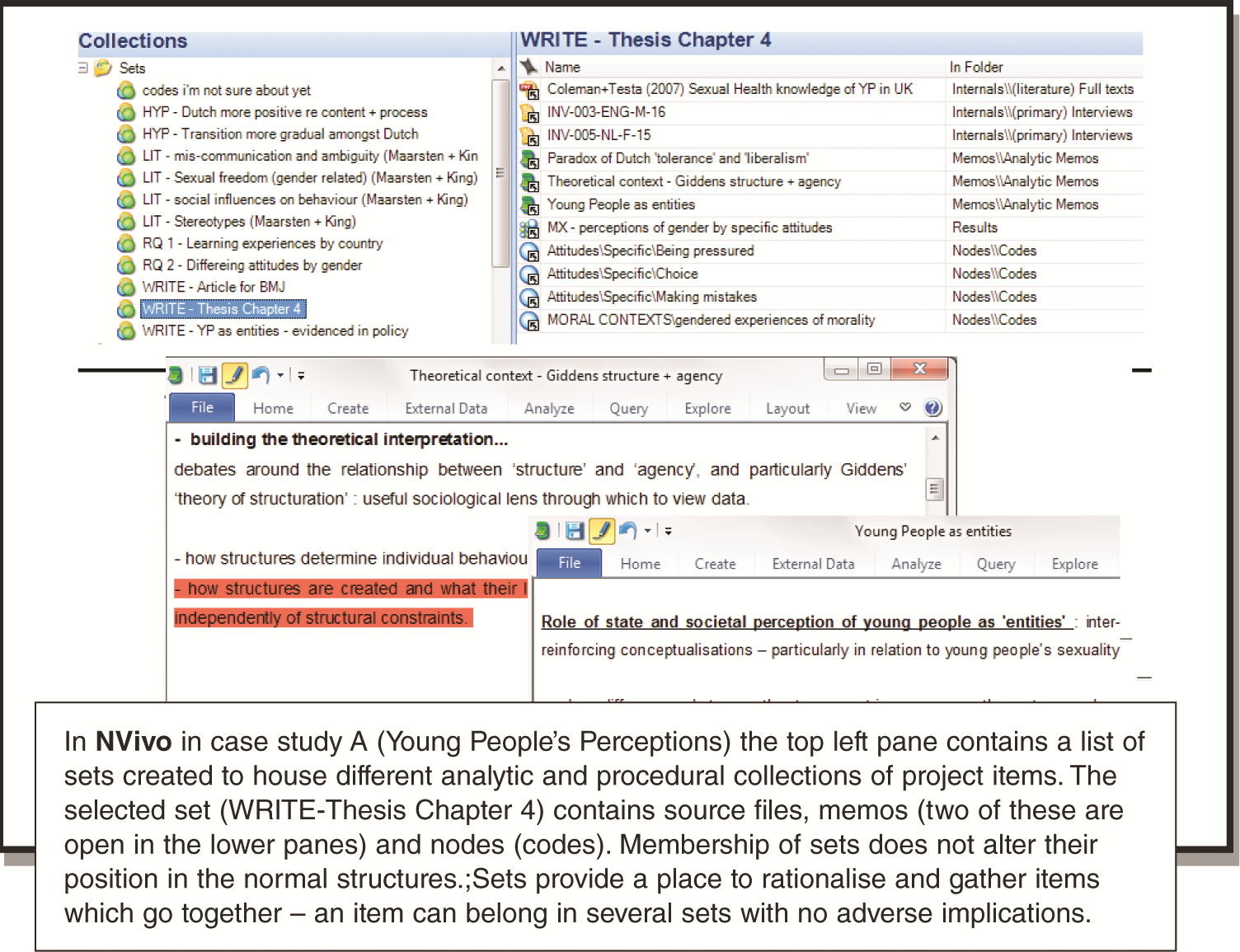Figure 10.7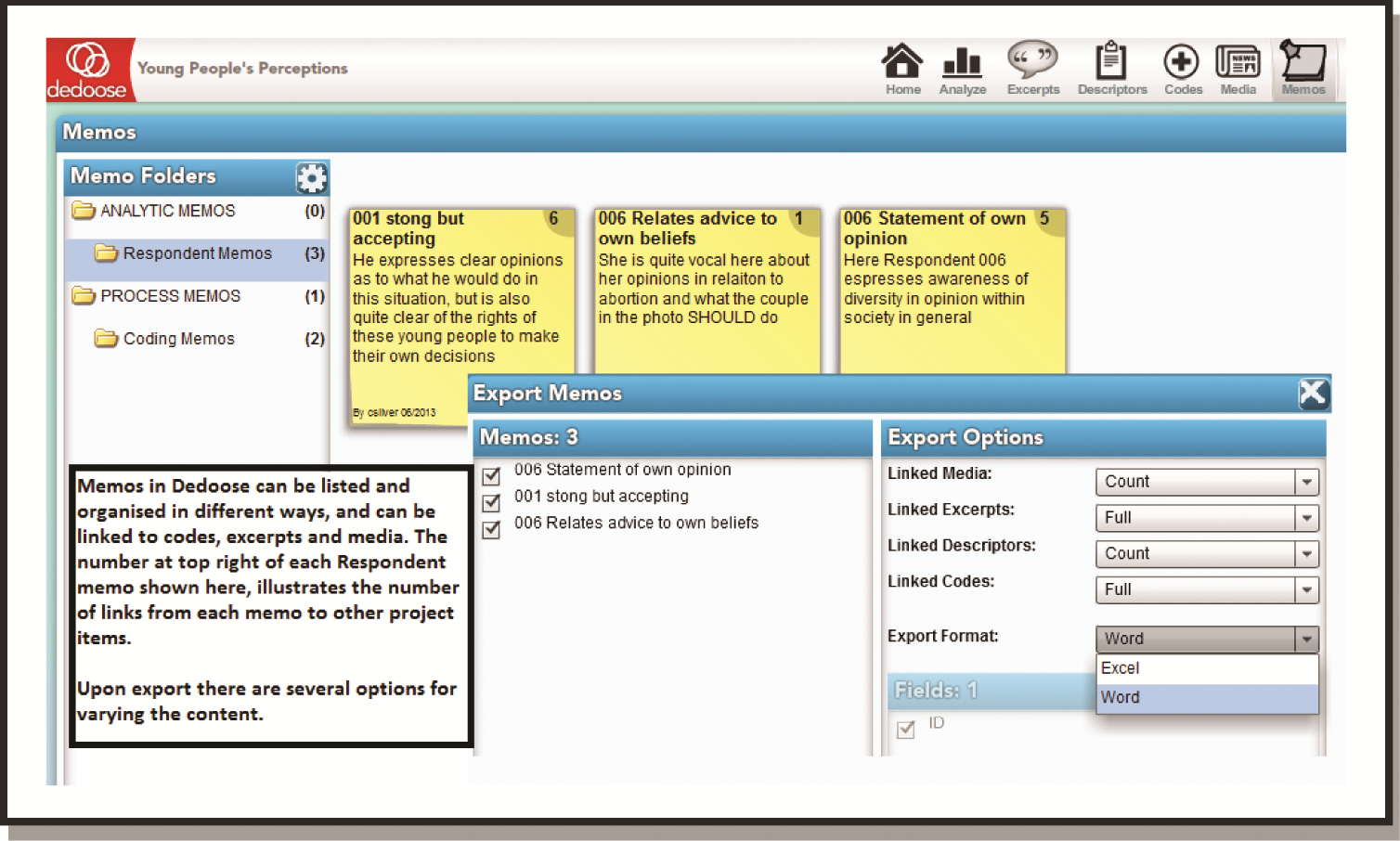Figure 10.8

## Chapter 11: Mapping Ideas and Linking Concepts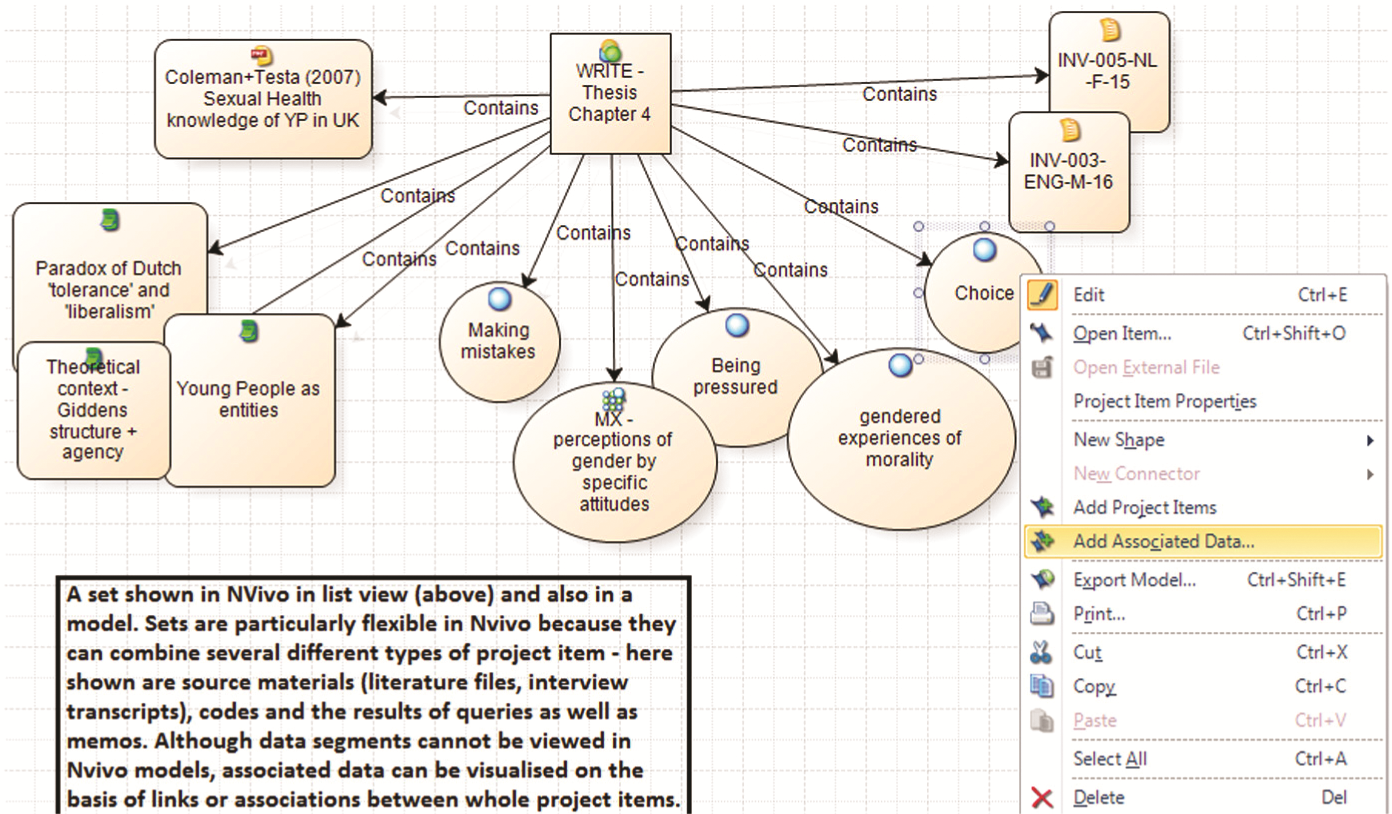Figure 11.1Figure 11.2Figure 11.3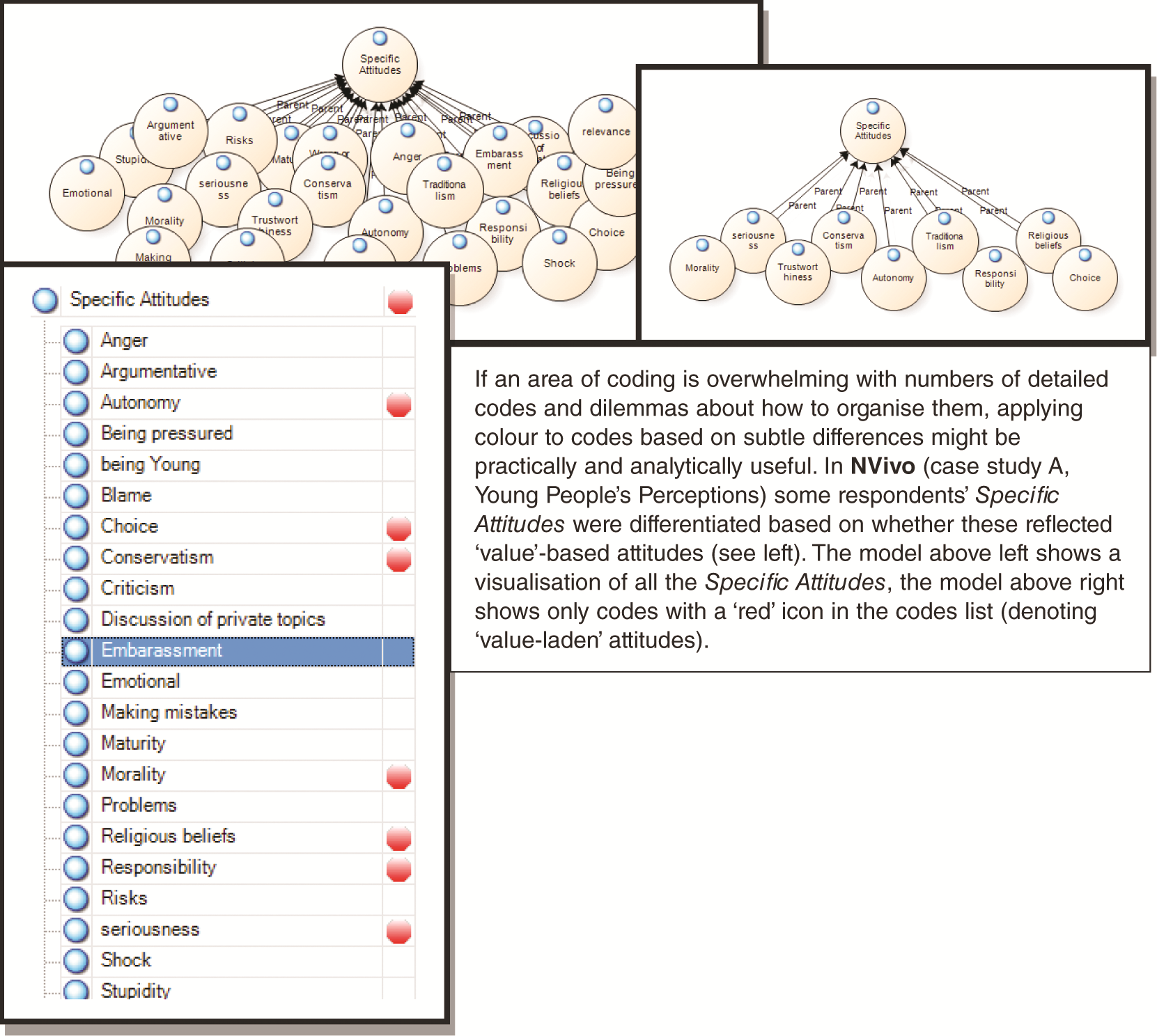Figure 11.4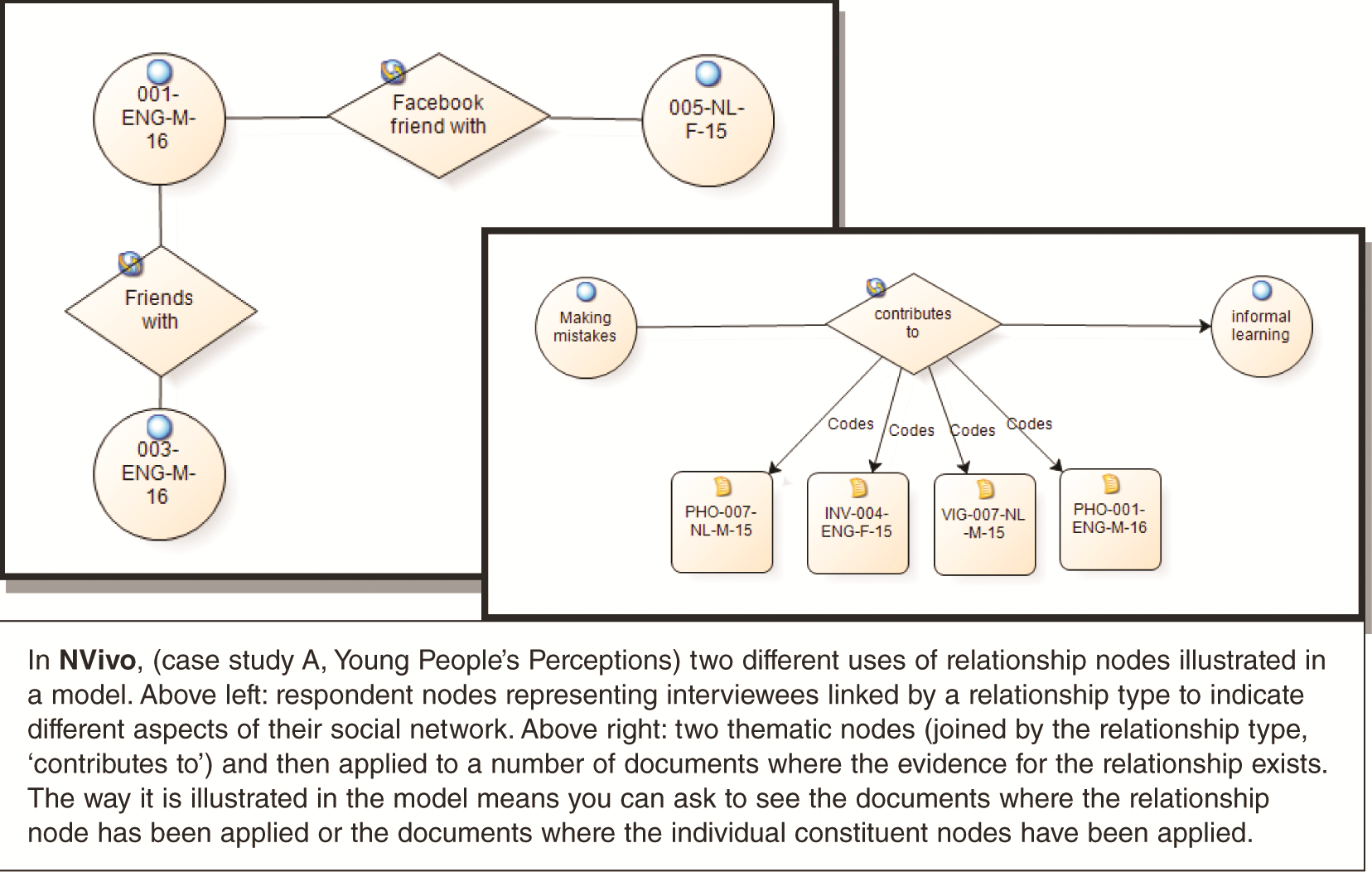Figure 11.5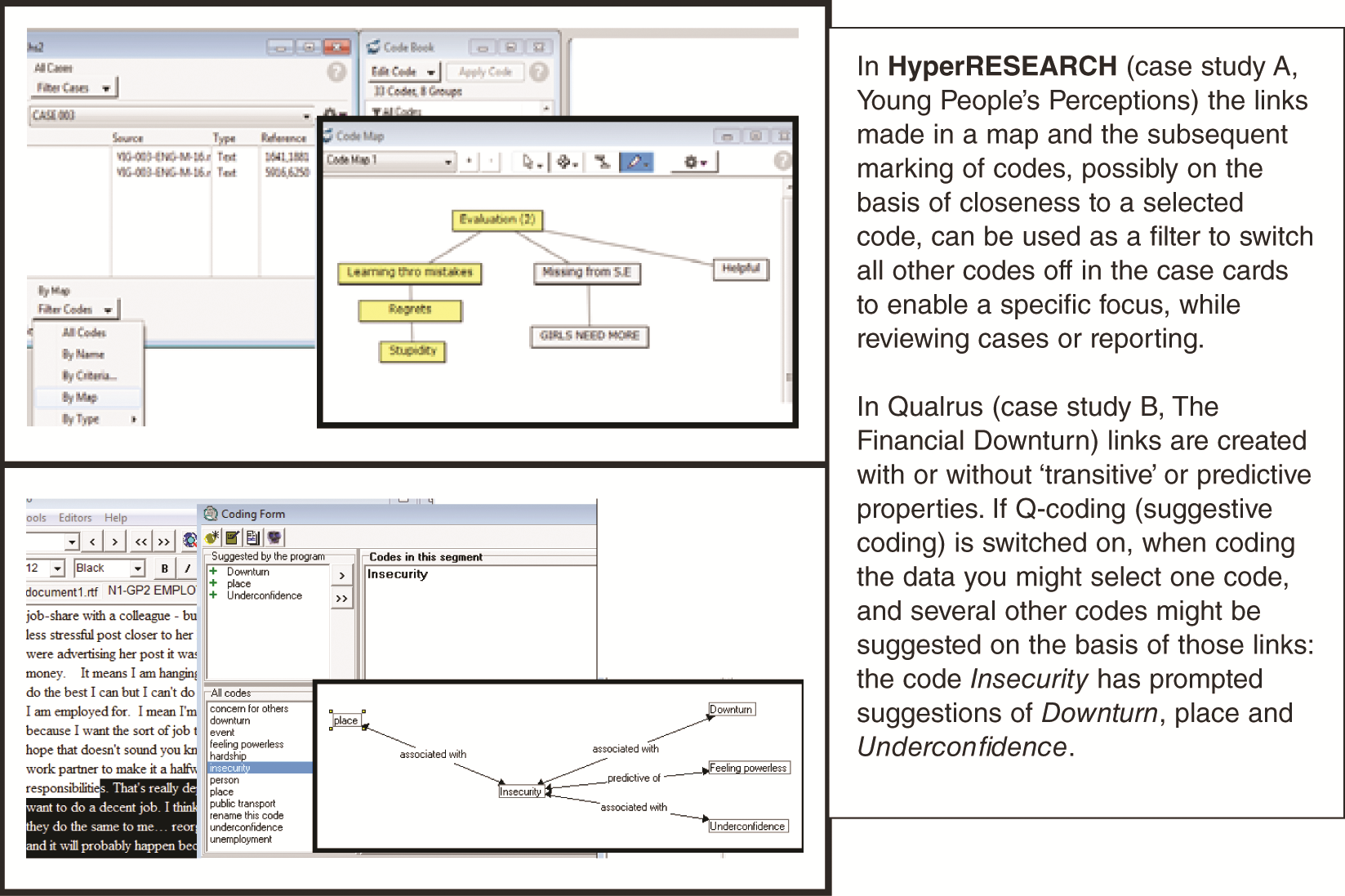Figure 11.6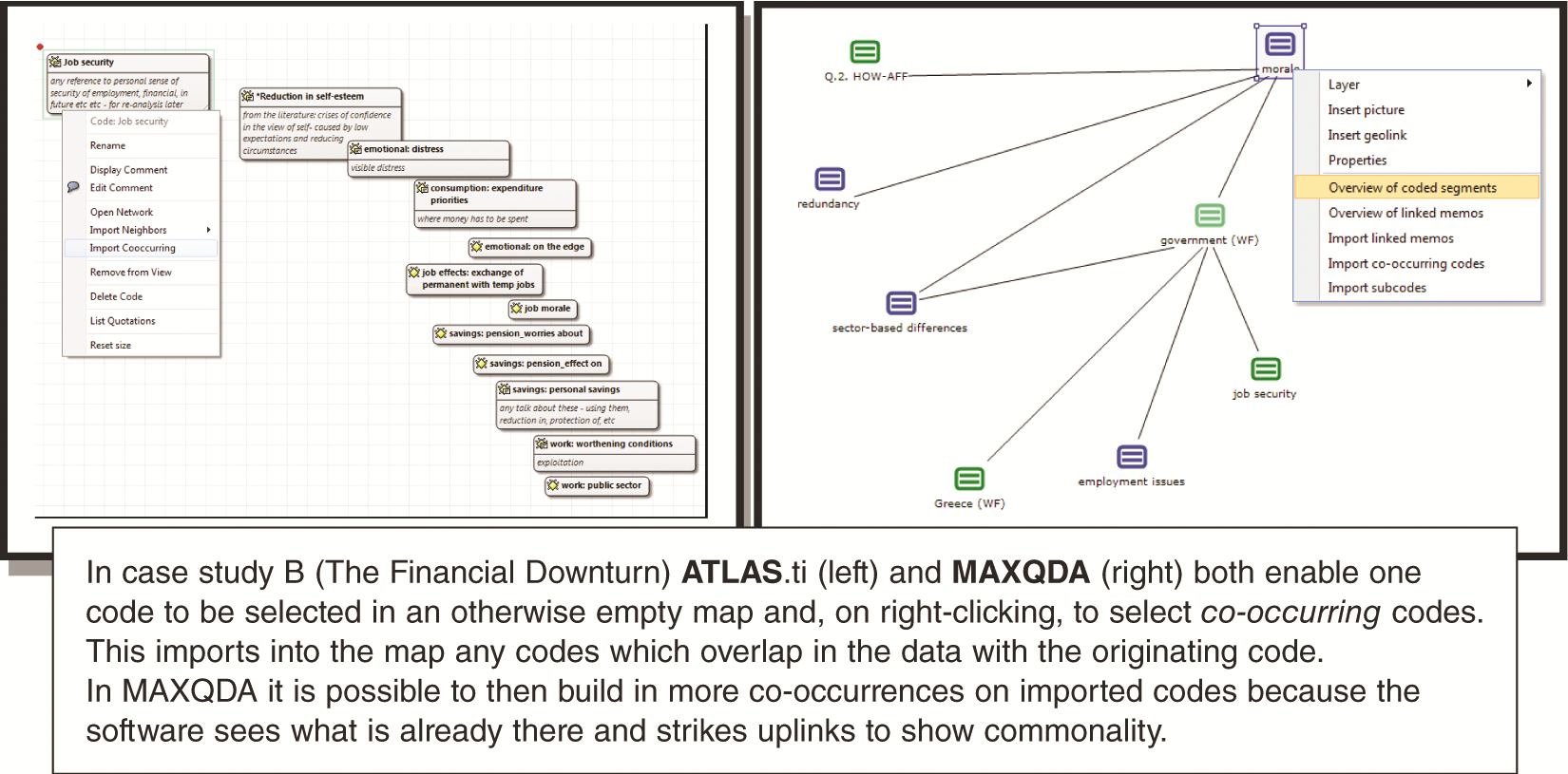Figure 11.7

## Chapter 12: Organising Data by Known Characteristics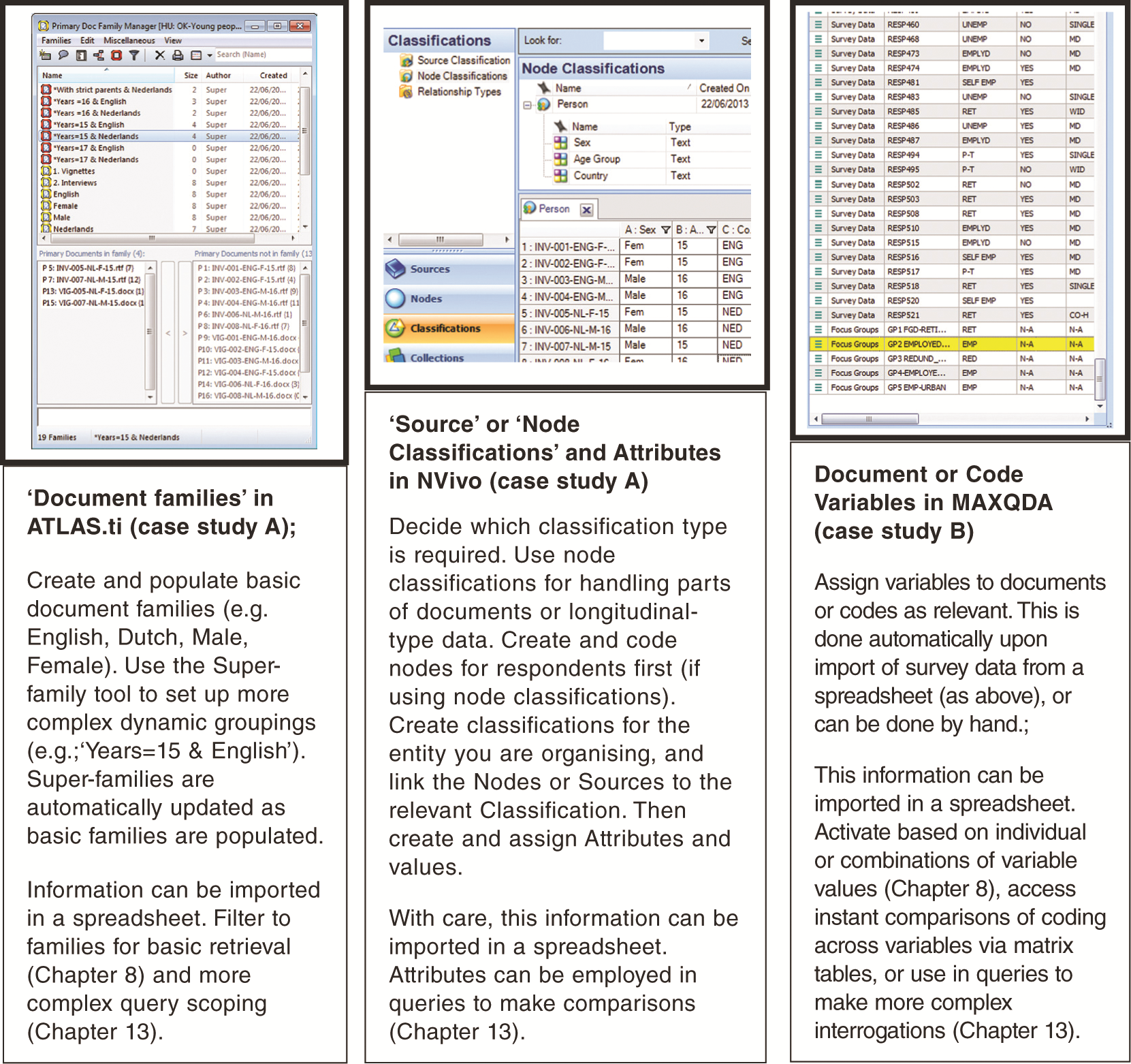Figure 12.1Figure 12.2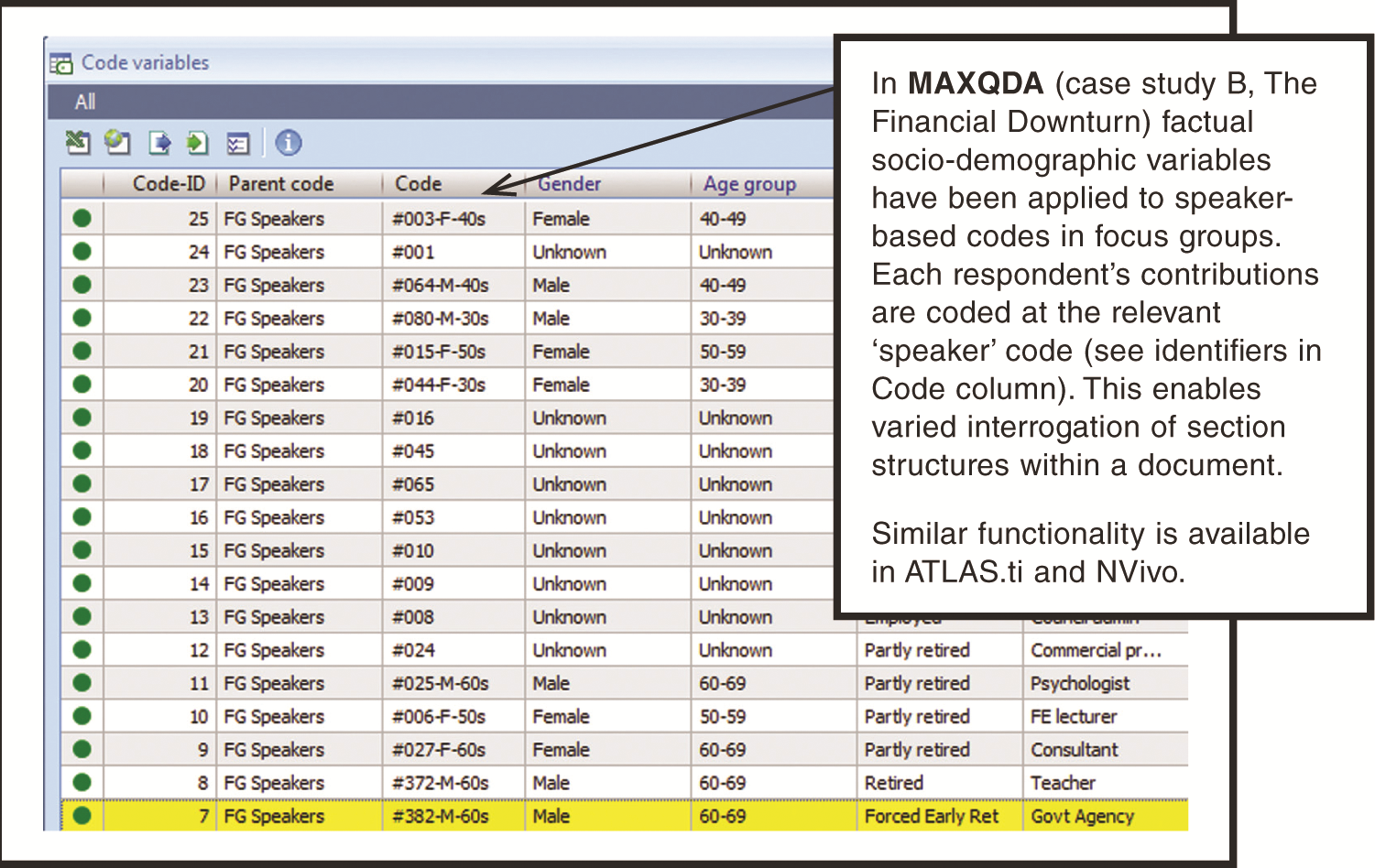Figure 12.3

## Chapter 13: Interrogating the Dataset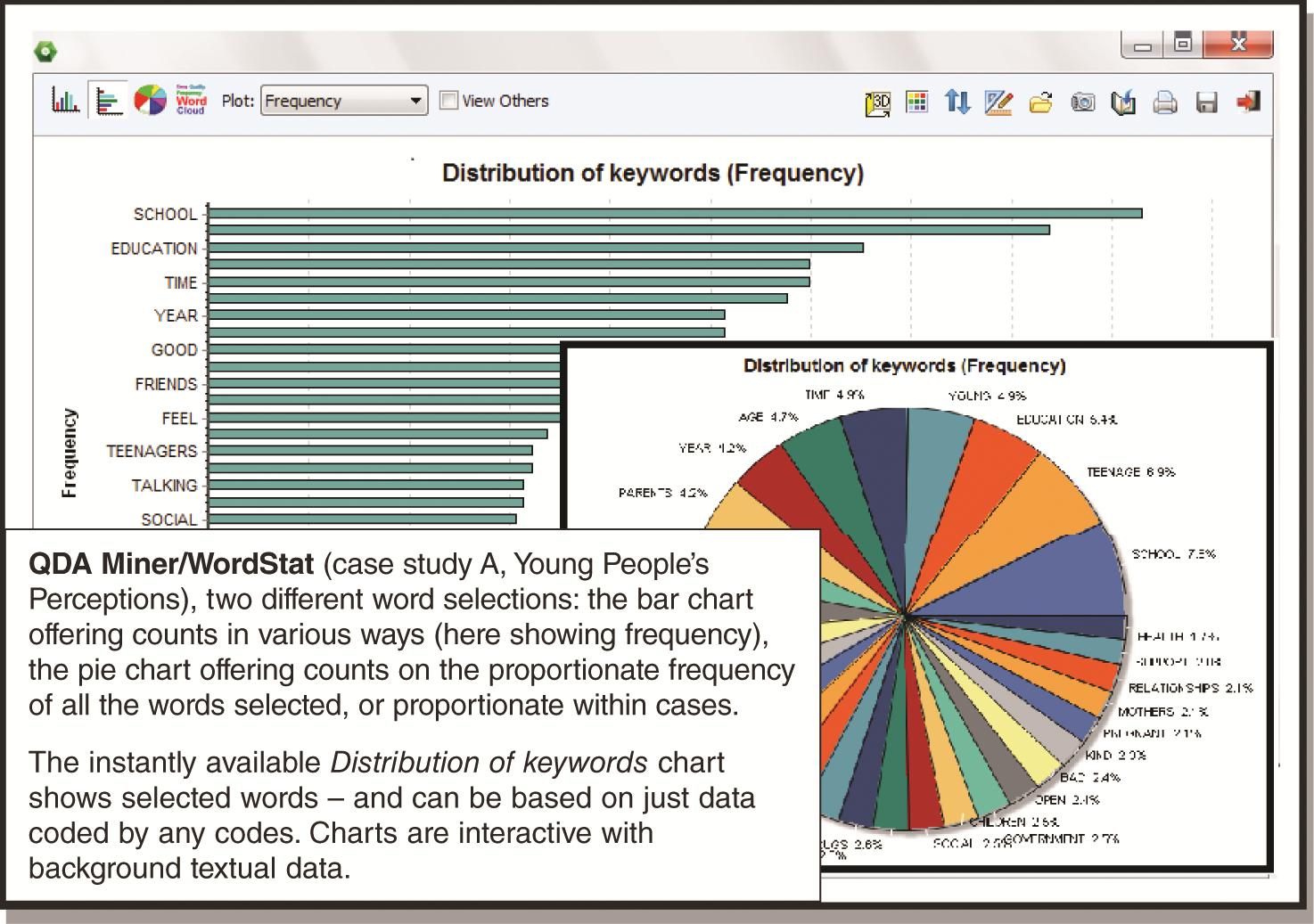Figure 13.1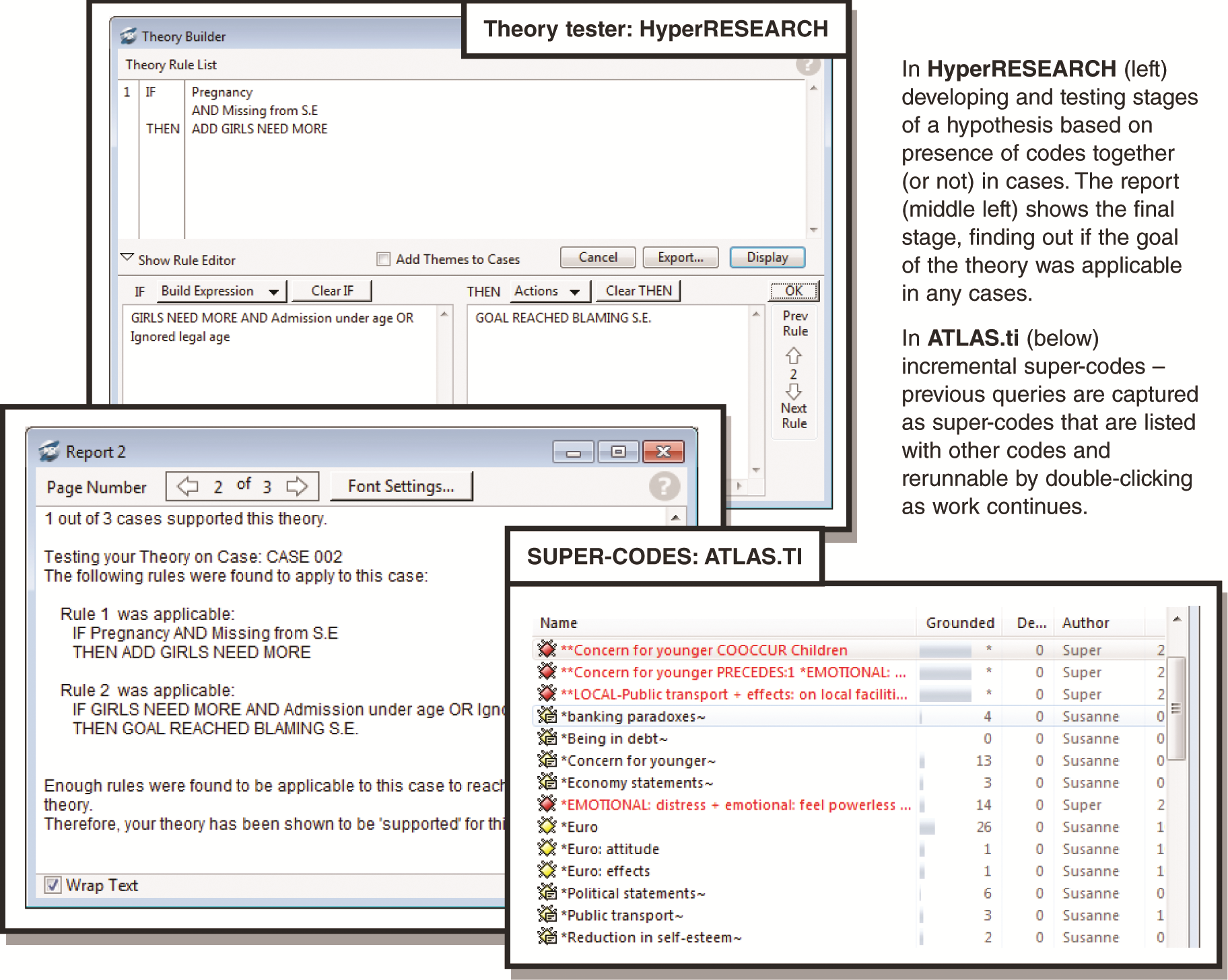Figure 13.2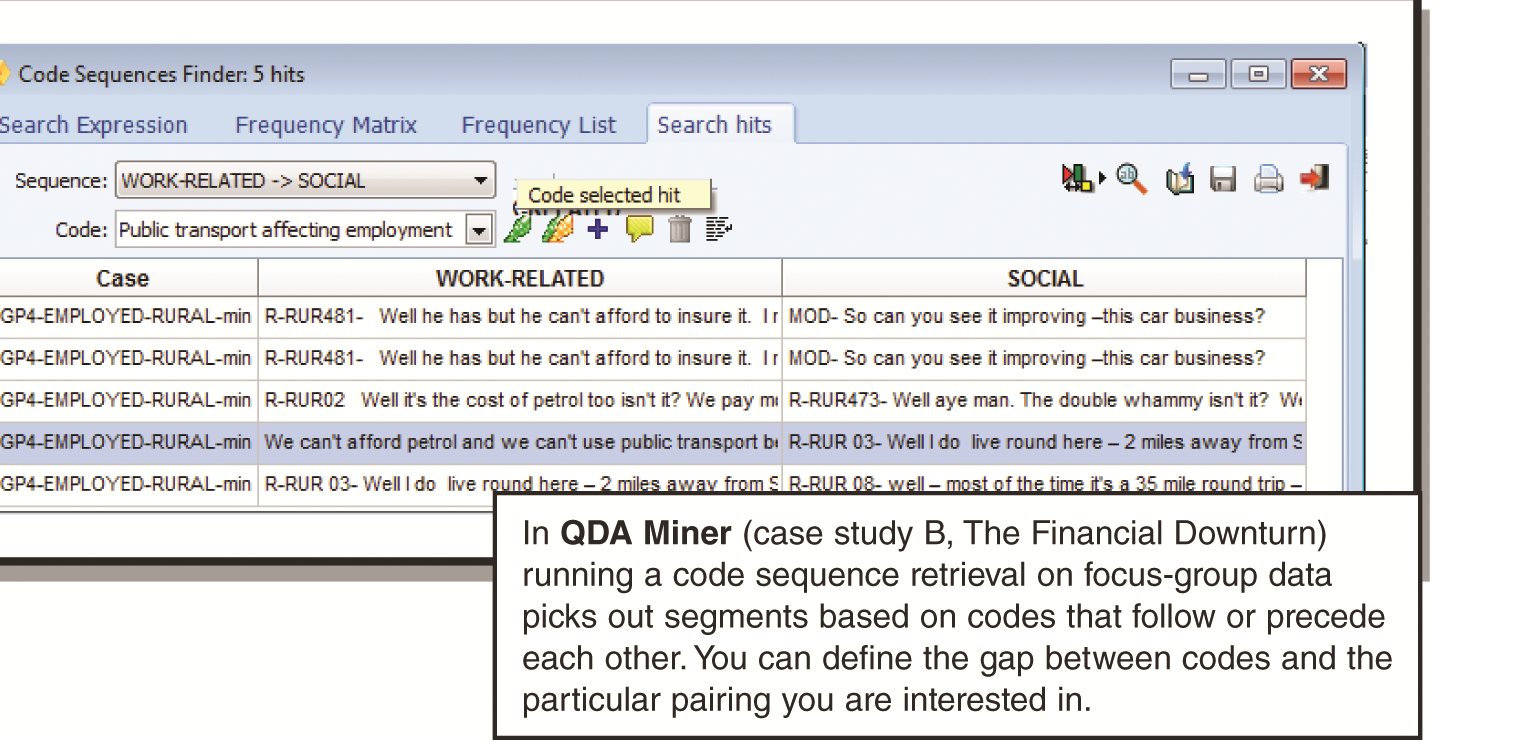Figure 13.3Figure 13.4Figure 13.5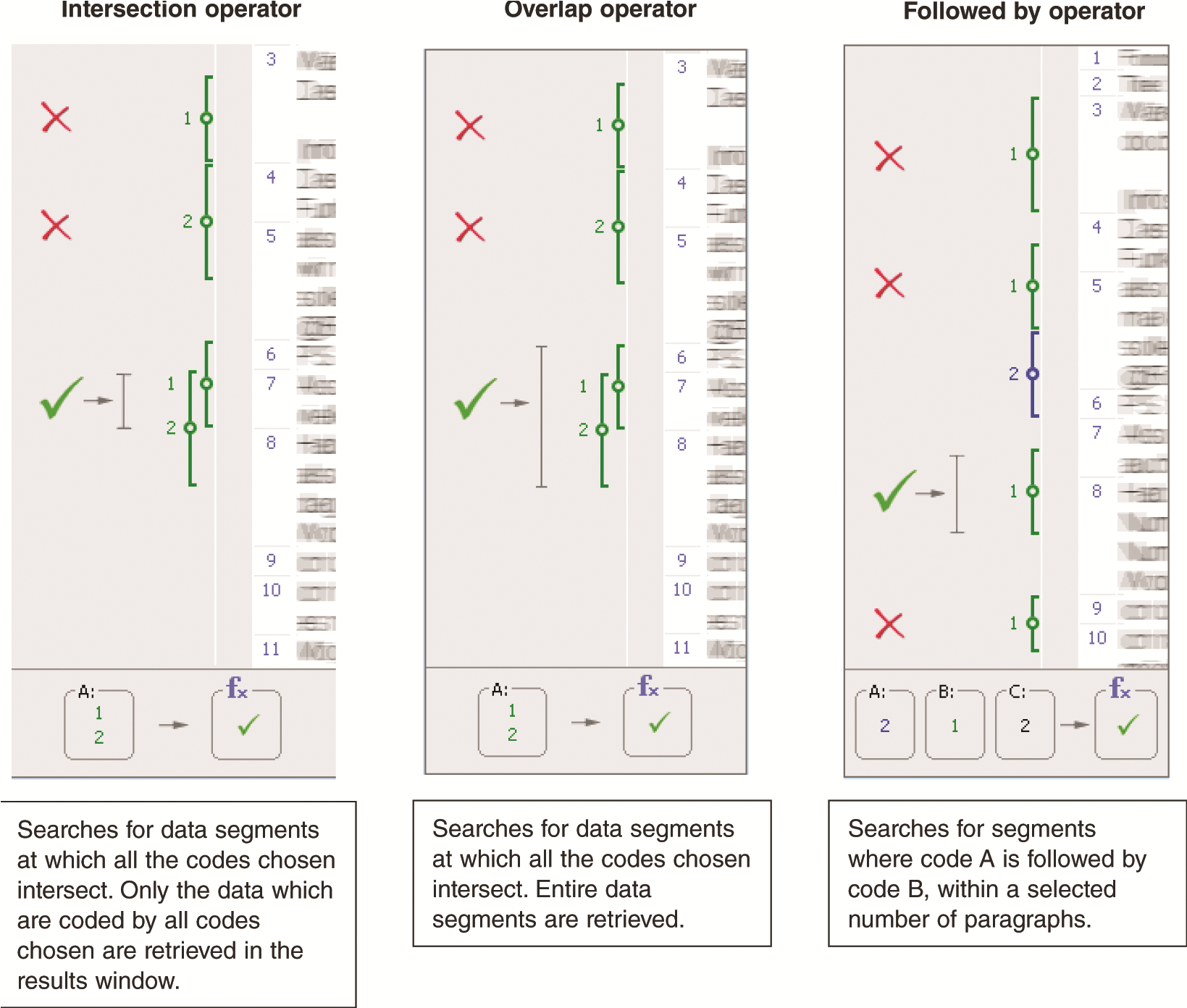Figure 13.6Figure 13.7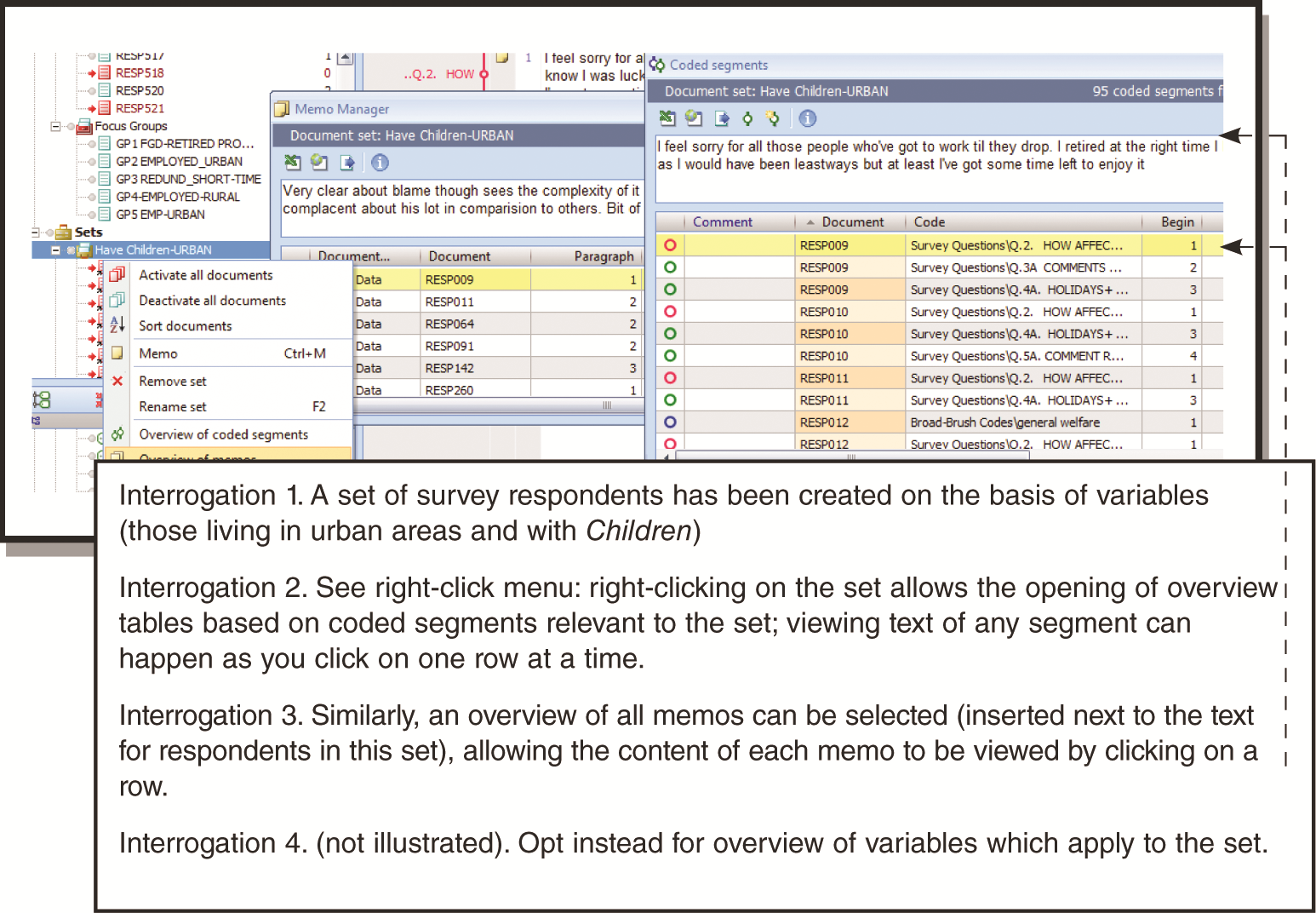Figure 13.8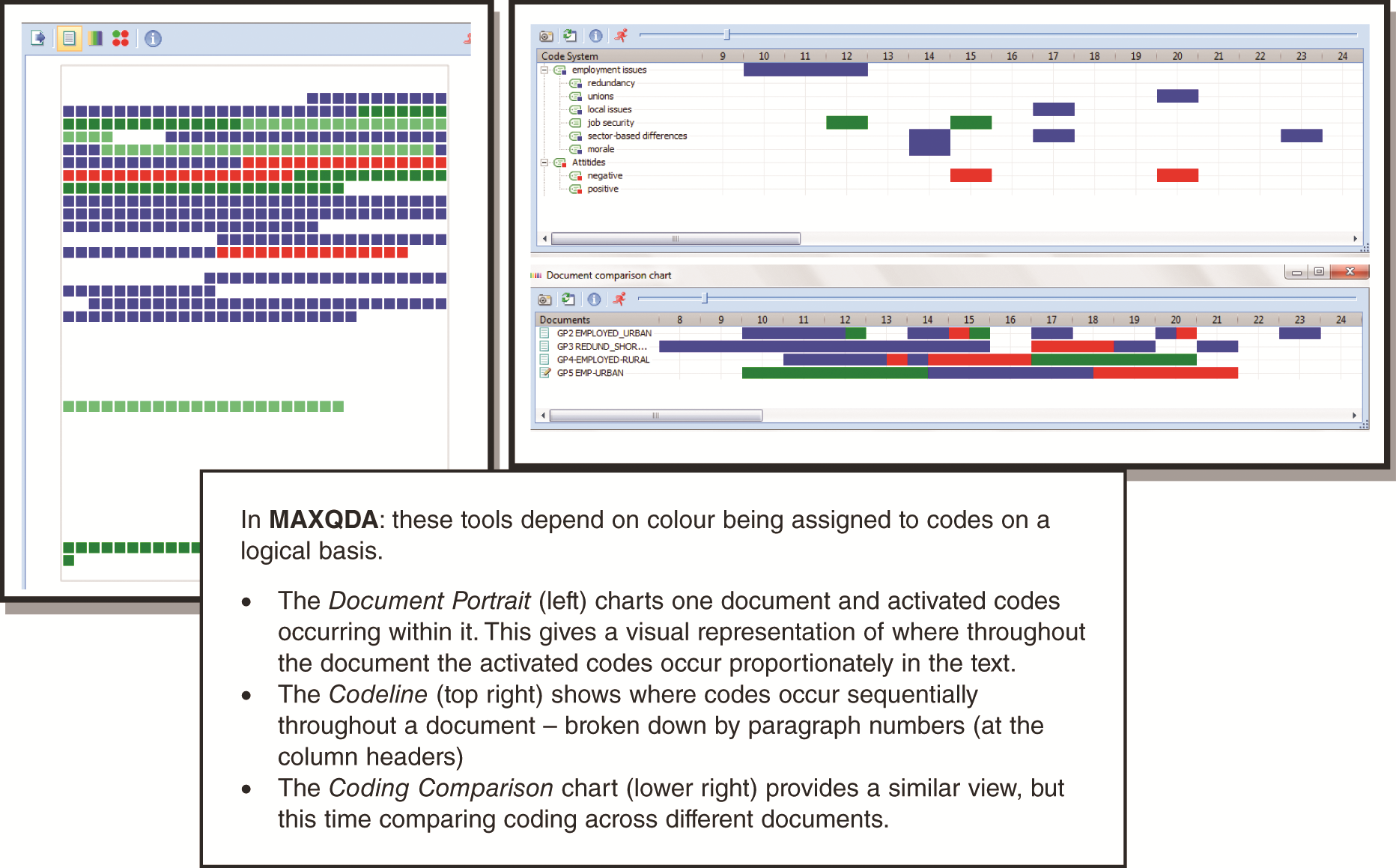Figure 13.9